入门_入门到精通 - CSDN
• Python入门基础教程：python教程旨在掌握python开发的入门基础用法与其核心操作，便于大家在短时间内可以使用python进行后续的工作与学习。课程内容包括Python环境搭建（Anaconda方式）、变量、List基础结构、List...机器学习 机器学习算法 机器学习实战 python机器学习
• 1.C#急速入门 2.C#之面向对象 观看地址：https://edu.csdn.net/course/detail/9447 3.C#高级进阶（期待中……） 4.程序员内功修炼 - 数据结构(C#) 观看地址：https://edu.csdn.net/course/detail/10505 5.Unity百家...c# c#语言 c#计算器
• 一门初级、从入门到精通的C语言C++语法教程，由毕业于清华大学的业内人士执课。从简单的HelloWorld入门程序，到深入的C语言C++核心概念，均为您娓娓道来，言之必详、听之必懂。让C语言C++编程变得简单，让C语言C++...c语言 c c语言程序设计 c语言指针
• 有点烦躁，来写篇文章把，也算是对自己过往的一个总结，也给想要入门计算机的人分享一点经验。如果你是一个初学者，我敢保证，读完这篇文章让你节省一年以上的时间。因为这是我本科两年时间的经验。 还是先要介绍...
    专栏开了大概有一个星期了，一直没有时间来写文章，也没有心情来写文章。现在是23:45，一个人坐在实验室里边挺凉的。有点烦躁，来写篇文章把，也算是对自己过往的一个总结，也给想要入门计算机的人分享一点经验。如果你是一个初学者，我敢保证，读完这篇文章让你节省一年以上的时间。因为这是我本科两年时间的经验。

还是先要介绍一下我自己，这样方便别人看文章的时候考虑文章有没有必要继续读。因为这篇文章对层次高一点的人来说，看起来是浪费时间的。所以先声明一下这篇文章仅供如入门者参考。顺便介绍一下我自己：我是在读本科大三，我很庆幸我选择了我喜欢的计算机专业，我也很庆幸我没有选错它，我是真的爱它，我只有一个女朋友，她叫DELL，她有时候会给我撒娇，比方说报个404的错误啊（使用struts2框架的时候刚入门，404很常见，哦对了，servlet的时候也会报404错误。我最感谢的就是一次次的飘红，一次次的报错，因为每一次都会让我加深对框架的理解，想要把错误解决掉，就一定要懂框架的执行流程。）她是最苛刻最严谨的，连一个英文字母错了，都不行。想要入门计算机这一行，最好是一个爱折腾的人，能够把折腾当做一种乐趣。如果你真的确定，你还爱她，并且发誓至死不渝，那就开始把！

到了大学，不能说像大多数人一样懵懂，我还算是比较成熟的，从大一就开始做了各种兼职，实现了个人的小康生活，因为生活费从来没给家里要过，也从来没花完过。貌似有点跑题了哈，咱是谈技术的，其实也不然，我先说一下自己的经历，总结一下自己大学两年以来的错误，希望经验能够给后边的人吸取到。我是本科大学，在青岛。一开始就过着忙碌的生活把，一遍边增加自己的生活的能力，一遍上课。两年以来，最大的错误就是天真的以为上课跟着老师上课好好学就够了，这个致命的错误，导致的结果是浪费掉至少一年的时间。希望读到这里的朋友，如果是本科生的话，一定要记住大学里边全靠自己，不管你的大学多么优秀。我们永远要以最高的标准要求我么自己。对于我们来说，大二下学期，大三上学期应该是技术大爆炸的时期，到了大三下，就可以再学学前沿一点的技术了。在风口浪尖上虽然风险大一点，但是机会也就多一点。所以有幸刷到我这篇文章的你，希望你能够早点入门，不要觉得跟着老师好好上课就够了，我们计算机行业需要培养很强的自我学习能力才行。课上好好学是必须的，课下自己去找一些好的资源，自己学，效果会很好的。

如果你有幸刷到我这篇文章，并且还能看到这里，那你一定是赚到了。接下来可全是干货了，分享一下自己的资源，学习方法。到了大二的暑假我才感受到了技术的魅力，和吸引力，以至于我暑假没回家，在宿舍自己鼓捣了一个半月，加强了前端，学习了JQuery，做出来一个小游戏，用JQuery插件实现各种小的功能，比方说淘上的放大镜，万花筒啊，手风琴啊，轮播图啊，炫酷的动态背景啊，等等。又稍微加强里一点java基础。这是我真正的接触到资源，就是有比较好的视频。学习这个，从书上看到的知识基本上都过时了。再说从书上获取知识，你要保证你学习能力极强，总结能力极强。前期入门的话还是去看一些好的视频，比人总结过得，学起来容易一点，也偏重点一些，我们要善于站在巨人的肩膀上才行。等你学到一定程度以后，别人总结的东西不够你吃的时候，可以淘一些好的书，去获取知识。

ps：我分享一套Java整个体系的课程（尚硅谷老师分享的，我在这里无偿分享出来，想走java体系的，这一套就够了，跟着学）

至于学习方法： 这是我自己的一篇关于学习方法的文章，希望可以帮到你

链接：https://pan.baidu.com/s/1W6HyhFG7kkrYKWkeQDeOsA
提取码：wu6h

当你有了资源以后，希望你能够好好的利用，不要浪费掉。跟着好好的学，靡有不凡鲜克有终，不积小流无以致江海。希望能够坚持下去，入门没有那么容易，在安装工具上会花费大量的时间，个人建议不管安装什么都先去看教程，跟着完整的教程安装，会让你事半功倍。一开始自己敲成来的程序，只有我和上帝能看懂，出了Bug以后，只有我自己能解决。我们需要锻炼独自解决问题的能力，一开始你可以把调试的错误复制下来到百度上去搜搜看，可能一开始比较花时间，但是慢慢的你会发现，你解决问题的能力就上去了，浏览帖子的时间明显低了。再慢慢的，你会发现国内的站已经不能满足你解决的问题了，可以翻墙去国外的站搜搜看。

千里之行始于足下，基础的东西最好掌握的牢固一点。但是是在不懂的东西，可以先过去，有些内容到了后边，就会自然而然的懂了。就想我一开始学习java基础。我死活不清楚泛型有什么用，后来跟着别人做项目，需要用到代码抽取了，泛型自然也就用上了，并且大发感慨，泛型太神奇了！太好用了，一开始基础很少接触到用法。一步一个脚印学下去，我相信，用不了三个月，你就对编程有点感觉了。半年，你就真的入门了。慢慢的跟着做项目，总结一些经验，自己试着敲一些代码出来。

都写到这里了，如果你还在看，我就把压箱底的最后的一点东西拿出来把，只是个人经验，在学习的过程中，一定要做好笔记，很多东西，你会发现，你遗忘的速度远远超过你记忆的速度，很多东西要学好多遍的，至于到熟练的掌握。就需要大量的练习才行。笔记的话个人建议做电子版的，一来不容易丢失，二来查阅起来方便，三来电子笔记要快很多，一个截图两秒能抵你写的三分钟。一开始我的笔记都在word上，只有自己能看，后来开始写博客，我发现博客上写笔记真的很方便，而且自己能看，别人也能看。有错误别人说不一定还能给你指出来。在学习的过程中做好备份，比方说你安装的软件啊，什么的。做好备份，我相信你肯定会有重装电脑的需求的，到时候不用下载，有备份直接就能安装，都是自己经常使用的版本，这样会方便很多。将自己的重要的代码收藏起来，一些重用率很高的代码不防写在博客上，方便以后自己查看使用。

最后的最后希望你能真的爱上你的女朋友。细心照顾她，别对她发脾气。作为程序员一定要有分享的精神，将自己的经验分享出来。一定要有工匠精神，对知识，对技术永远不满足，永远充满渴望，你要知道，一旦选择计算机这个行业，就一定意味着学习是一辈子的事了。你永远都有学不完的东西。临渊羡鱼不如退而结网。

今后会持续的分享我个人的经验、教训、总结。刚入门的话可以继续关注我，谢谢。大家可以交流。（希望半夜赶出来的文章对初学者能有帮助）

深夜了。我要回去睡觉了，明天还有课。

***增加（强列推荐读 下边的链接文章，同样是我自己写的）

下边这篇文章给出了具体的学习计划，根据我的经验，我觉得成长就是在用时间犯错，然后换取经验。

这个计划写于上边文章一年之后

大学生如何走在别人的前边，即使非985，211。

更新时间：2019.05.08

如果觉得上边的内容还不够，可以看看下边的一张图，我今天得到的，我觉得它给我查漏补缺了！

由于图片过大，有点畸形了，建议下载下来看。（看到图片，直接点击就可以放大）

链接：图片链接
提取码：tgri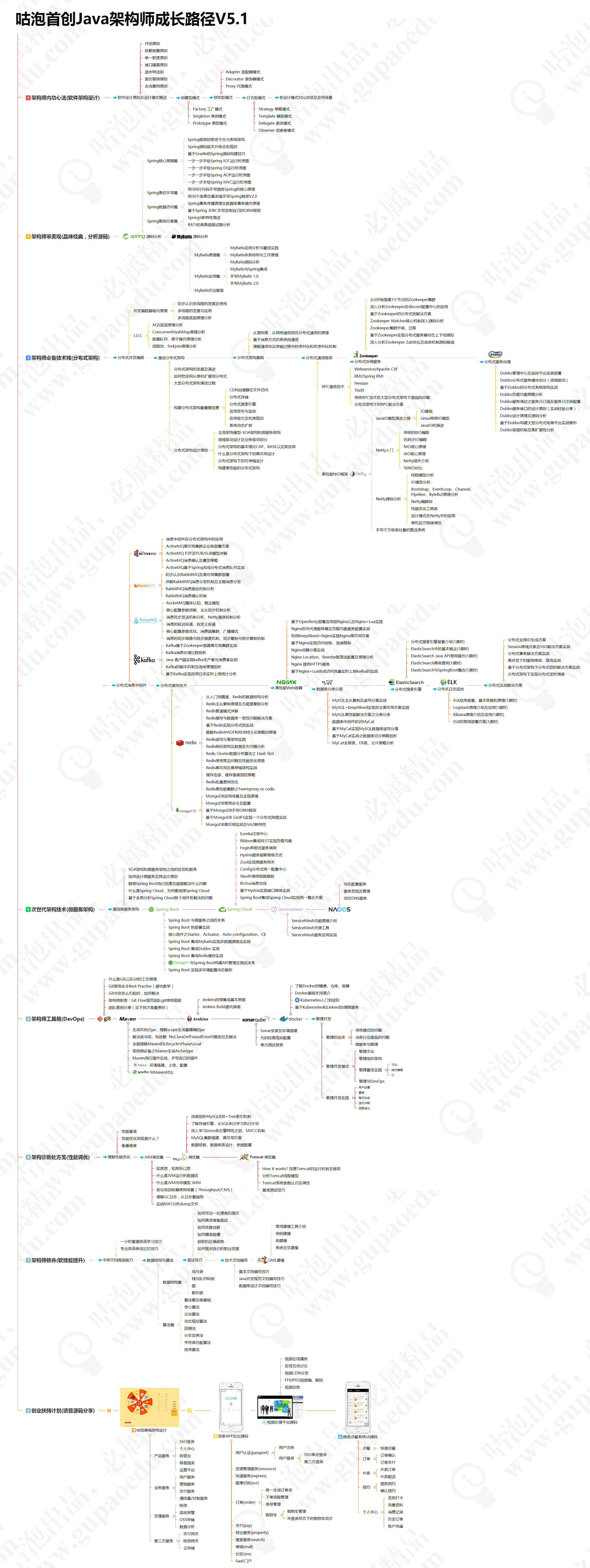展开全文• 引言 最近也有很多人来向我"请教"，他们大都是一些刚入门的新手，还不了解这个行业，也不知道从何学起，开始的时候非常迷茫，实在是每天回复很多人也很麻烦，所以在这里统一作个回复吧。 Java学习路线 ...
引言
最近也有很多人来向我"请教"，他们大都是一些刚入门的新手，还不了解这个行业，也不知道从何学起，开始的时候非常迷茫，实在是每天回复很多人也很麻烦，所以在这里统一作个回复吧。
Java学习路线
当然，这里我只是说Java学习路线，因为自己就是学Java的，对Java理当很熟悉，对于其它方面，我也不是很了解。
基础阶段
首先是基础阶段，在基础阶段，我们必须掌握Java基础，Mysql数据库，Oracle数据库，JDBC，Linux基础，Java8新特性，数据结构和算法，设计模式。
对于基础阶段，我们所要掌握的就是基础，虽然是基础，但学起来也够呛。因为是初学者，所以基础阶段会有一点难度，但只要肯坚持，这些也没什么难的。对于Linux、数据结构、算法和设计模式我们掌握一些基础就行了，也没必要学得非常透彻。
Web基础
基础阶段过后，我们还必须要掌握一些Web方面的知识，Html，JavaScript，JQuery和AJax，同样的，对于这些知识，我们只需掌握基础，而不需要深究，否则，你将耗费大量的时间。
JavaWeb
JavaEE
最后是JavaEE阶段，当你学到这个阶段，你对Java的程序开发已经了如指掌。然而，自己开发的过程是艰难而复杂的，所以JavaEE阶段我们需要去学习一些非常优秀的框架，来帮助我们进行程序开发。
对于框架，我们需要学习Struts2，Hibernate，Spring，SpringMVC，JPA，SpringData，MyBatis，Spring Boot，Spring Cloud，Dubbo。要学习的框架其实非常多，有些框架也十分有难度，我们在学习阶段只要能够熟练使用它们即可，有能力的话可以研究一下源码，看看框架的底层实现。当然，除了框架，我们还需要学习Maven，Git，Github，ActiveMQ，Jenkins等。
在JavaEE阶段学习的东西是最多的，但如果你经历了JavaEE阶段的学习，你的编程水平将会有质的飞跃。
其它
这里是我在写了这篇文章几天后才加上的，有人向我反映，说数据结构和算法不学好就只能当个码农，说我在文章中指出"只需学习数据结构和算法的基础即可"是误导别人。确实，这句话是没说错，我在之前的文章中也强调过数据结构和算法的重要性，它们是程序的灵魂。然而，数据结构和算法是具有一定难度的，对于新手可能非常地不友好，新手要想彻底掌握它们是很困难的，所以我只是说在基础阶段掌握基础即可，而不需要去深究。而随着学习的深入，你的知识体系将会越来越庞大，这个时候，对于数据结构和算法可不能仅仅停留在表面了，我们应该去更加深入地研究这些东西。
在这里也感谢大家给我提出的意见，只要大家说的在理，说的是对的，我都会去及时改正。金无足赤，人无完人，在写文章的过程中，难免会有一些不太严谨的地方，这里也欢迎大家批评指正。
学习资源
我知道，对于有些名词，你们可能都没有听过。没关系，按照这个路线一点一点地往下学，相信你会感受到编程的魅力所在。
下面对整条知识路线进行一个梳理，并附上对应的学习资源，需要的小伙伴们下载就好了。

基础阶段
Java基础——链接：链接：https://pan.baidu.com/s/17yh-M7LYSRsXmFeQKSrAww  提取码：mqf4
Mysql数据库——链接：https://pan.baidu.com/s/1NfS6Q9fb_OMna608w9GYeg 提取码：94ns
Oracle数据库——链接：https://pan.baidu.com/s/12uVSvaY4pbXRWy2DtIIf8w 	提取码：5r8p
JDBC——链接：https://pan.baidu.com/s/1QF9S_GpucFvoLSrQqV6d0Q 	提取码：ltnh
Linux基础——链接：https://pan.baidu.com/s/1e0ekEVjmtfNWAEO0123wDw 		提取码：pwxz
Java8新特性——链接：https://pan.baidu.com/s/103nPGgEEjbxzuy1rHRJepg 	提取码：6omt
Java数据结构和算法——链接：https://pan.baidu.com/s/1LQ__Hp7i-TR7gRa4YJQXJg  提取码：zwwd
Java设计模式——链接：https://pan.baidu.com/s/1ik7PRwlAVgJzhSCdthdu1A 	提取码：nr5e
Web阶段
JavaScript DOM——链接：https://pan.baidu.com/s/1_HtKFXeRm5xF7p2vYk_c7w 提取码：ks6g
JQuery——链接：https://pan.baidu.com/s/1IPPNu2qsJ-MgLX7mGcVYbQ 提取码：umdr
AJAX——链接：https://pan.baidu.com/s/1MC1iryOq9_SSV1qWYk5P5Q 提取码：78hu
JavaWeb
JavaWeb阶段——链接：https://pan.baidu.com/s/1EJYIgAuAHhaJLv8Pp-QjgA 提取码：584f
JavaEE
JavaEE阶段全套视频——链接：https://pan.baidu.com/s/1rJ5MyvFMuN_cbDVbw-2B3A 	提取码：mdlf

课件及源代码
文章发布过后，有很多人来问我有没有源码和课件，这里当然是有的，为了方便大家，我就把源码和课件链接放在这里，也不用劳烦大家一直问了。
链接：https://pan.baidu.com/s/18fr2-Hy-0aoRr9wDIl1zbg 提取码：2zxp
关于Java学习路线上的所有资源都在这里了，欢迎大家下载！
马上就要到中秋佳节了，在这里也提前祝贺各位中秋节快乐，身体健康，阖家欢乐！
2020年5月14日更新
因为百度网盘自身的一些原因，导致这些资源经常出现失效的现象，而且资源数目比较多，维护起来比较麻烦，所以我特地将所有资源进行了一个整合，上面的所有学习资源都在这个链接里面：

链接：https://pan.baidu.com/s/12SnRLQnt8xf8taE1Km893A
提取码：hrey


展开全文学习资源 Java 导航
• 真小白入门之Github ---之前入门python的时候真的是千辛万苦的踩教程的坑，起初写python入门教程一个是为了记录另一个是为了可以帮到其他需要帮助的人。在真的接到大家被帮助的评论的时候真的炒鸡开心，github我也...
真小白入门之Github
-----之前入门python的时候真的是千辛万苦的踩教程的坑，起初写python入门教程一个是为了记录另一个是为了可以帮到其他需要帮助的人。在真的接到大家被帮助的评论的时候真的炒鸡开心，github我也刚刚开始入门，希望能帮到一同不停踩坑又打开这篇博客的你（篇幅较长，谨慎踩雷）！
-----至于Github的介绍我觉得没有什么比百度更全面的了，都要入门了总该知道这是干啥的吧（莫名觉得自己很厉害的样子٩(๑❛ᴗ❛๑)۶）
-----然后我是真的真的第一次接触github所以有什么不对的地方请多多指教！

####一、注册
-1.打开https://github.com/
打开就是个注册页面，我英文不太好（如此理直气壮ヾ(◍°∇°◍)ﾉﾞ），如果恰好你也英文不太好的话可以用谷歌自带的翻译，很好用。我的谷歌因为其他的一些工作需要必须用低版本的目前翻译不了（其实也不知道是不是因为版本低），如果又恰好你的也不能用谷歌翻译，就用火狐自己下个翻译插件，哪里不懂点哪里（当然能好好学英语更好了）密码需要至少包含一位小写字母，至少七位
-2.点完sign  up for GitHub就到这个（验证账户）页面-3.点击create an account（注册填入的邮箱这时应该也收到邮件了）用我超凡的英语能力翻译一下解释一下这个页面的选择项
单选选项：
Unlimited private repositories for KaTeX parse error: Expected 'EOF', got '#' at position 1019: …，到这里我们真的注册成功了。
#̲###二、在github上创建… ssh-keygen -t rsa -C “你注册github时的邮箱地址”
这里真的真的有重点，我真的真的踩了很多坑！
ssh-keygen后面有空格
-t后面有空格
rsa后面有空格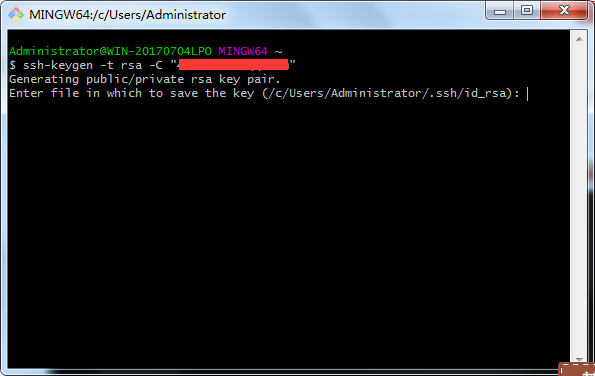Enter passphrase (empty for no passphrase):
Enter same passphrase again:
接着呢就会提示你输入密码，按两次回车就可以了我们不输入密码，这样我们每次提交或者下载代码的时候就不用输入密码了，当然你也可以输入密码，这样每次操作都需要密码（注意这个密码跟你登录github的密码是不同的哦）
2.出现下面的界面就是告诉你你的key存在某某路径，就是你设置的那个路径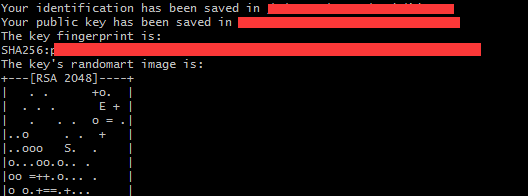3.我是存在默认路径下，现在我们就去找到它4.打开id_rsa.pub，复制里面的全部内容
5.打开我们的github，鼠标移到头像的地方，选择settings6.选择红框圈中的SSH and GPG keys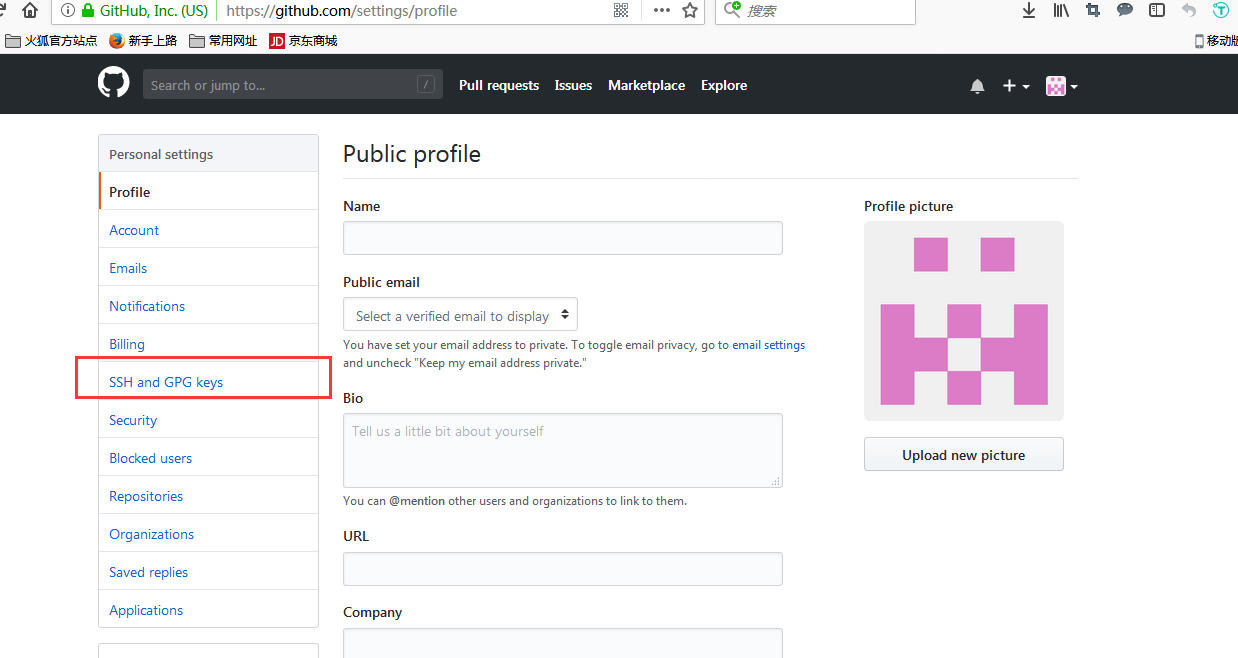7.选择new ssh key，创建一个新的key9.这样我们就将本地库与github库用ssh key连接起来了
下图就是我们添加的key（没错我的key就叫keyone(～￣▽￣)～ ）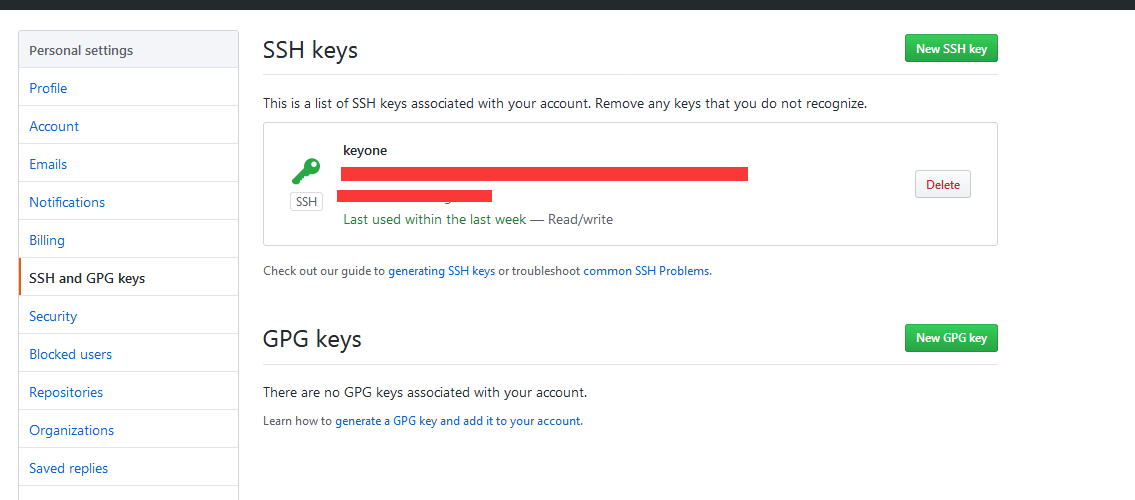连接起来以后我们就要测试一下是不是真的连起来了
####5.测试ssh key
1.输入 ssh -T git@github.com  （注意不是输入自己的邮箱哦）
2.接下来会提示你
The authenticity of host ‘github.com (xxx.xxx.xxx.xxx)’ can’t be established.
RSA key fingerprint is SHA256:xxxxxxxxxxxxxxxxxxxxxxxxxx.
Are you sure you want to continue connecting (yes/no)?
输入yes即可
3.如果你设了密码的话会提示输入密码吧，如果和我一样没有设密码就会出现以下提示You’ve successfully authenticated, but GitHub does not provide shell access这样就成功了4.输入
git config --global user.name “你github的名字” （注意前边是“- -global”，）
git config --global user.email “你的github邮箱地址”
5.接下来就要操作把我们一开始在github建的库搞到本地，方便我们进行操作
5.1、我习惯把我的东西放在f盘，所以我在f盘建立了一个文件夹专门存储在github下载下来的库，输入f:/Github，这个自由选择哦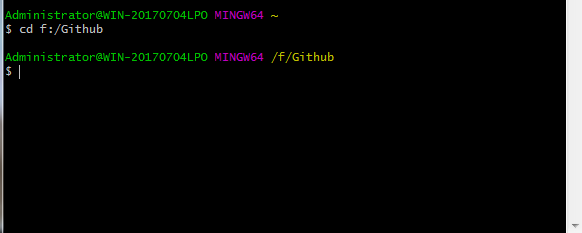5.2、然后我们去github把我们创建的库的地址复制下来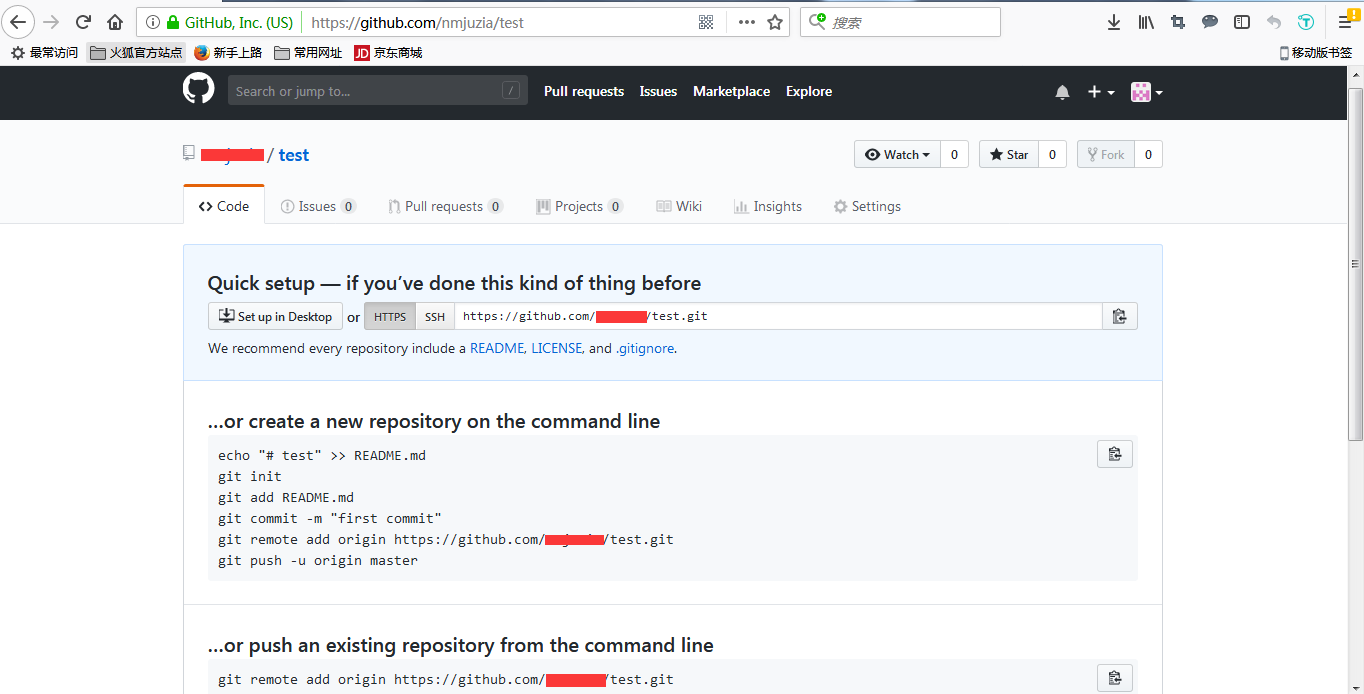5.3、输入git clone https://xxxxxxxxxxx  （后面是你复制下来的地址）
5.4、这样我定位的F盘Github文件夹下就有个叫test的文件夹了，这个其实就是一开始我们在github创建的那个叫test的库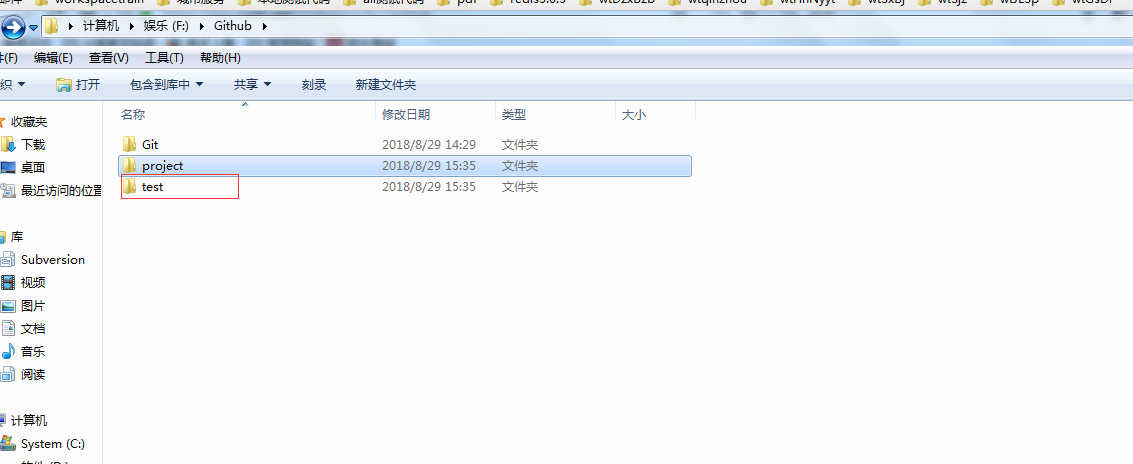6.我们可以试着在这个文件夹里创建一个文件并上传到我们的github的test库中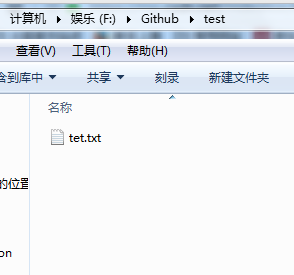6.1、我们将文件位置定位到test中，然后输入ls来查看test文件夹中有什么文件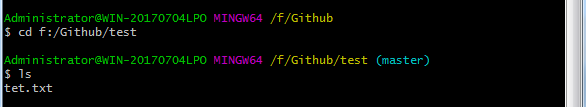可以看到有我们创建的文本文档tet.txt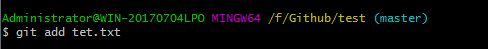6.3、输入 git commit  -m “注释”（这里因为我之前创建了一个叫tet的所以我又创建了一个叫tes的文件夹）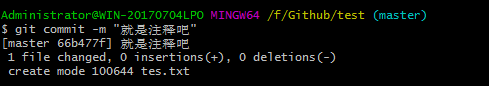6.4、输入git push origin master，会出现输入邮箱地址的提示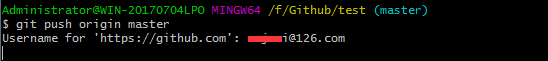输入后会弹出输入密码的框，输入github的密码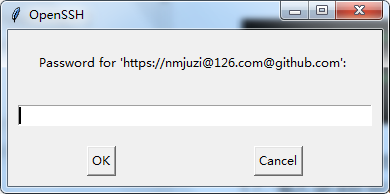就会出现以上图中的提示，这时候我们就成功把文件提交到github上了
7.我们一起去github看看我们从本地上传到github的文件
如果你还停留在某个页面因为英文不好不知道点哪里，一头雾水找不到我们的库的时候，点下图的小黑猫就可以然后我们就到了好像我们主页的地方的样子，就看见左下角的小红框处有我们一开始创建的那个叫test的库，是它是它就是它我们的英雄小哪吒！单击后我们就到了这个页面，发现了我们在本地上传的tes.txt以及我瞎写的注释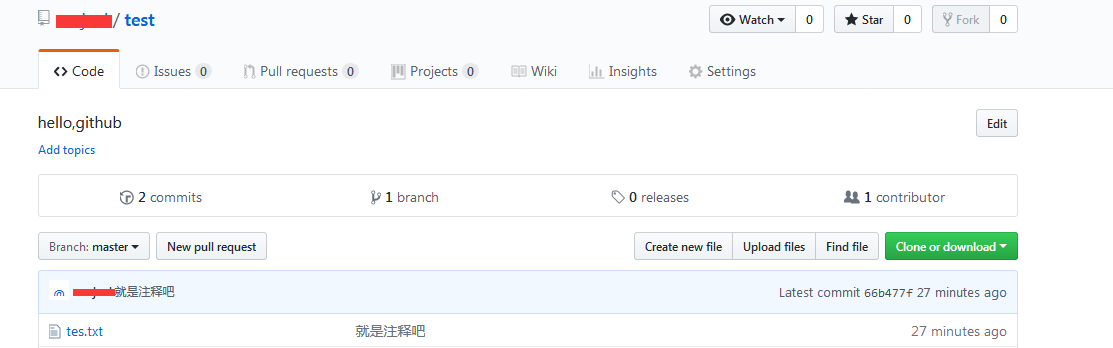8.这时候其实我们真的成功了，我们可以总结一下
以后我们就先创建一个库把它档到本地，然后将我们的代码放到库的对应的文件夹中，然后git add ，git commit -m "注释注释注释啊 " 、最后git push origin master，我们就能将本地的代码提交到github库上了
至此这篇教程就结束了，我是第一次接触github，所以可能会有很多不对的地方呀(oﾟ▽ﾟ)o  ，你可以批评我但是不可以骂我，嗯，标题的真小白当然就是指的我啦，希望大家愉快的入门


展开全文• LaTex简介 TeX 是由Donald Knuth创造的基于底层编程语言的电子排版系统(TEX是Honeywell公司在1980年为其Text Executive文本处理系统注册的商标，它与 TeX是两回事)。TeX能够对文档的排版进行非常精细的操作，...
LaTex简介
TeX  是由Donald Knuth创造的基于底层编程语言的电子排版系统(TEX是Honeywell公司在1980年为其Text Executive文本处理系统注册的商标，它与 TeX是两回事)。使用TeX可以对版面做精细的操作，生成精美的文档。TeX提供版面编辑的底层命令，但是使用时操作复杂，工作效率不高. TeX允许用这些底层命令定义出可以执行复杂操作的扩展命令.  LaTex是由Leslie Lamport开发的的TeX扩展命令集合。LaTexj通过整合常用的版面设置操作，降低排版的工作量和难度。 LaTex 强大的排版功能，特别是对科技文档的支持，已经使它成为撰写科研论文的事实上的标准。
TeX系统生成的是DVI(Device Independent)文件。随着pdf文件格式的流形，LaTex在支持DVI文件格式的同时也可生成PDF格式文件。
TeX在不同的硬件和操作系统上有不同的实现版本。目前Unix/Linux上常用的TeX系统是teTeX，Windows中有MiKTeX和fpTeX。CTeX是中文套装。下面仅简要介绍在使用LaTex中一些常见问题。
LaTex 与 Word
Word 是微软公司推出的一款文档和文字处理软件，它也具有较强的排版功能。与LaTex相比，Word最大优势是其"所见既所得"的特点，因而入门门槛低。另一方面Word的语法和拼写错误检查等能力比LaTex强。
LaTex作为一款排版系统，格式控制、公式编辑方面比Word好用。LaTex生成的文档比Word文档更美观。LaTex的入门门槛比较高，使用LaTex不仅要编辑文本，整理文档格式，还要处理编译过程中出现的bugs，如果使用中文，更要注意GBK,UTF-8等文档编码这些琐碎问题。另外LaTex做的幻灯片没有Word做出来的好看。
LaTex 和 Word两款软件针对的用户群体不一样，在文字处理和文档排版方面有不同的理念。 两者在功能上有许多重合之处，各有优势和不足。
LaTex 命令架构
Tex环境中，字符，图片等内容都放在隐形的盒子(box)容器内。这些盒子根据Tex和Latex的默认规则以及相关命令粘结成更大的盒子。字符组合成单词，单词组合出语句。语句在放入页面盒子时，根据版面格式分解成行列。
版面格式布置盒子在页面中的位置，字符等文档内容的位置是由它们在盒子中相对坐标确定。例如，一个字符盒子如下图所示。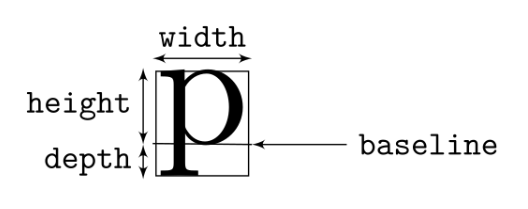width是盒子的宽度。基线(baseline)将盒子分割成上下两部分。height和depth分别确定这两部分的高度。 字符盒子基于基线相互粘结。
LaTex提供不同层次的命令帮助用户设置文档版面。它最底层是大约300个TeX基础命令，例如 \hbox， \vskip等。
LaTex的核心是一组建立在TeX基础命令上的宏命令，例如 \documentclass, \usepackage, \begin.等。
再往上就是类文件，例如foo.cls等。当\documentclass{foo}时，就是加载foo.cls文件。
再往上是扩展包, 例如pic.sty等。我们可以使用\usepackage命令加载扩展包。
安装
Latex的衍生版本众多，中文环境中常用的时CTex。下载地址为
http://www.ctex.org/CTeXDownload

Ctex套装中自带Latex编辑器WinEdt
LaTex文件格式简介
TeX文件样例
下面是一个TeX文件的简单样例：
This is an example of TeX file format.

An example of mathematical formulaion: $ds = \sqrt{dx^2 + dy^2}$
\bye

编译说明：
前提： 已经正确安装TeX编译环境，例如：LaTeX
操作步骤：

将上述内容保存在一文本文件中，例如: foo.tex.
在Windows控制台环境中，使用

tex foo.tex

既可生成相应的foo.dvi文件。
LaTex文件样例
下面一个样例展示了LaTex文件的基本结构:
\documentclass[12pt, letterpaper]{article}
\usepackage[utf8]{inputenc}
\usepackage{comment}

% Title
\title{Document Title}
\author{Nobody \thanks{Somebody}}
\date{Some Date}

\begin{document}

\begin{titlepage}
\maketitle
\end{titlepage}

\tableofcontents

\begin{abstract}
This is a simple paragraph at the beginning of the
document. A brief introduction about the main subject.
\end{abstract}

First document. This is a simple example, with no
extra parameters or packages included.

\begin{comment}
This text won't show up in the compiled pdf
this is just a multi-line comment. Useful
to, for instance, comment out slow-rendering
while working on the draft.
\end{comment}

\end{document}

LaTex文件结构
一个LaTex文件分为两个部分：导言和正文。 在\begin{document}和\end{document }之间的是文档的正文内容。 在\begin{document}之前的命令称为preamble(导言)。 在preamble中通常定义文档的格式、语言等。上文所示LaTex文档其结构如下图所示: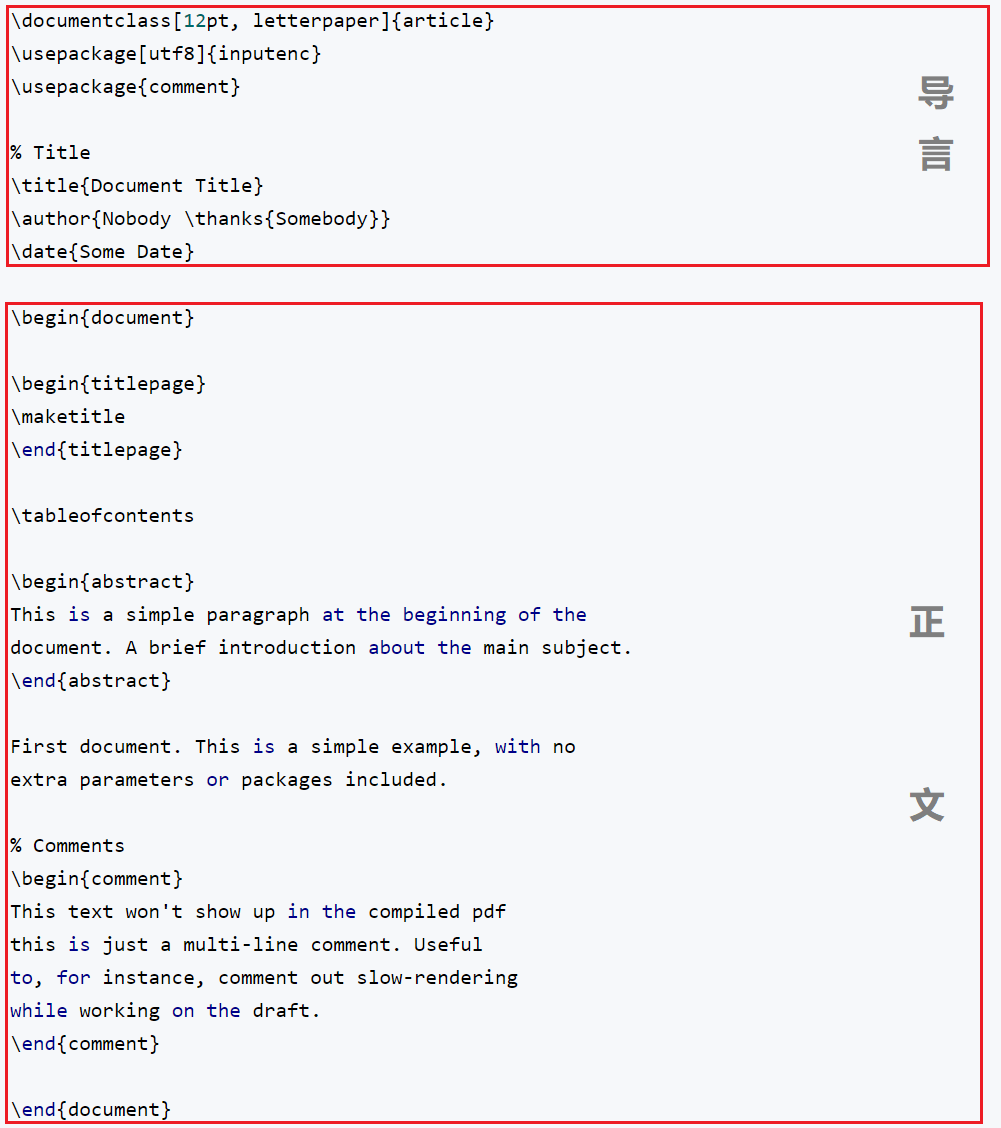文件拆分
当文档内容的结构复杂，需要分别保存在多个LaTex文件时，可以使用\input 或\include命令构建文档结构。例如:
\input{filename1}

或者
\include{filename1}

"\input{filename} imports the commands from filename.tex into the target file; it’s equivalent to typing all the commands from filename.tex right into the current file where the \input line is."
"\include{filename} essentially does a \clearpage before and after \input{filename}, together with some magic to switch to another .aux file, and omits the inclusion at all if you have an \includeonly without the filename in the argument. This is primarily useful when you have a big project on a slow computer; changing one of the include targets won’t force you to regenerate the outputs of all the rest."
"\include{filename} gets you the speed bonus, but it also can’t be nested, can’t appear in the preamble, and forces page breaks around the included text."
导言部分 LaTex命令简介
preamble(导言)部分通常用于定义文档的格式、语言等。常用的LaTex命令主要有\documentclass，\usepackage等。下面分别对几个常用的命令做简单介绍。
\documentclass
\documentclass命令是用于设置LaTex文件所生成文档的格式. 其命令语法如下所示：
\documentclass[options]{class}

常用的文档格式有:

Class
简介

article
科技论文，报告，软件文档等

IEEEtran
IEEE Transactions 格式.

proc
法律文书

report
长篇报告(如:学位论文)

book
书籍

slides
幻灯片

memoir
传记

letter
信件

beamer
PPT

文档格式修正项

Options
简介

12pt
文档正文使用的字体大小(默认为10pt)

a4paper, letterpaper
页面规格(默认为letterpaper或a4paper)

fleqn
Typesets displayed formulas left-aligned instead of centered.

leqno
Places the numbering of formulas on the left hand side instead of the right.

titlepage, notitlepage
Specifies whether a new page should be started after the document title or not. The article class does not start a new page by default, while report and book do.

twocolumn
Instructs LaTeX to typeset the document in two columns instead of one.

twoside, oneside
Specifies whether double or single sided output should be generated. The classes article and report are single sided and the book class is double sided by default. Note that this option concerns the style of the document only. The option twoside does not tell the printer you use that it should actually make a two-sided printout.

landscape
Changes the layout of the document to print in landscape mode.

openright, openany
Makes chapters begin either only on right hand pages or on the next page available. This does not work with the article class, as it does not know about chapters. The report class by default starts chapters on the next page available and the book class starts them on right hand pages.

draft
makes LaTeX indicate hyphenation and justification problems with a small square in the right-hand margin of the problem line so they can be located quickly by a human. It also suppresses the inclusion of images and shows only a frame where they would normally occur.

例如:
\documentclass[12pt, a4paper]{article}

意思是正文字体大小为12pt, 页面规格是A4, 使用article文档格式.
\usepackage
\usepackage命令设置在编译LaTex文件时要导入的扩展包，例如：
\usepackage[utf8]{inputenc}

\usepackage{comment}

封面格式
\title{Document Title}
\author{Nobody \thanks{Somebody}}
\date{Some Date}

设置所要生成文档的封面内容: 文档名，作者，日期等(这只是设置了封面格式，生成封面的是\maketitle命令)。
注释
% Comments

从百分号%开始到这一个行结束的部分是LaTex文件的注释内容，不在编译后生成的pdf文档中显示。
在\begin{comment}和\end{comment }之间也不在编译后生成的pdf文档中显示。
保留字符
# $% ^ & _ { } \  这些字符(reserved characters)在Latex中有特殊的意义，要想在生成的文档中显示这些字符，Latex文档中这些字符前加反斜杠"$\backslash$" : \# \$   \%   \^   \&   \_  \{   \}

因为两个反斜杠"\\"在Latex中是换行命令，可使用
$\backslash$

输出反斜杠$\backslash$
~波浪线(tidle)在LaTeX中是插入空格命令，可用数学公式环境的$\sim$向TeX文档中插入波浪线.
正文部分LaTex命令模块简介
生成封面
\begin{titlepage}
\maketitle
\end{titlepage}

按照在preamble中设置的封面格式生成文档封面
生成目录
\tableofcontents

生成文档目录
页码
设置页码数字类型
\pagenumbering{digit type}

其中digit type有：

arabic      阿拉伯数字(1,2,3,4)，默认样式


roman      小写罗马数字(i,ii,iii,iv)


Roman     大写罗马数字(I,II,III,IV)


  alph         小写拉丁字母(a,b,c,d)


  Aiph         大写拉丁字母(A,B,C,D)


设置页码可使用
\setcounter{page}{page number}

如果想让当前页不标页码，可使用
\thispagestyle{empty}

例如，如果在封面不标记页码，目录页使用小写罗马数字标记页码，正文部分使用阿拉伯数字标记页码
\begin{document}

%% Making title pate
\begin{titlepage}
\maketitle
\thispagestyle{empty}
\end{titlepage}

%% Contents
\pagenumbering{roman}
\tableofcontents

\newpage
\pagenumbering{arabic}
\setcounter{page}{1}

版面设置
空白
下面是LaTex插入空格命令小结
\,    inserts a \thinspace (equivalent to .16667em) in text mode,
or \thinmuskip (equivalent to 3mu) in math mode;
\!    inserts a negative \thinmuskip in math mode;
\>    inserts \medmuskip (equivalent to 4.0mu plus 2.0mu minus 4.0mu) in math mode;
\;    inserts \thickmuskip (equivalent to 5.0mu plus 5.0mu) in math mode;
\:    is equivalent to \> (see above);
\enspace   inserts a space of .5em in text or math mode;
\quad      inserts a space of 1em in text or math mode;
\qquad     inserts a space of 2em in text or math mode;
\kern <len>      inserts a skip of <len> (may be negative) in text or math mode (a plain TeX skip);
\hskip <len>   (similar to \kern);
\hspace{<len>}   inserts a space of length <len> (may be negative) in math or text mode (a LaTeX \hskip);
\hphantom{<stuff>}   inserts space of length equivalent to <stuff> in math or text mode.
Should be \protected    when used in fragile commands (like \caption and sectional headings);
\     inserts what is called a "control space" (in text or math mode);
(直接键入空格) inserts an inter-word space in text mode (and is gobbled in math mode).
Similarly for \space and { }.
~     inserts an "unbreakable" space (similar to an HTML &nbsp;) (in text or math mode);
\hfill    inserts a so-called "rubber length" or stretch between elements (in text or math mode).
Note that you may need to provide a type of anchor to fill from/to;

段落行间距
使用{setspace}扩展包. 例如
\documentclass[UTF8]{article}
\usepackage{setspace}

\begin{document}

%%双倍行间距
\begin{spacing}{2.0}
段落内容。
\end{spacing}

%%单倍行间距
\begin{spacing}{1.0}
段落内容。
\end{spacing}

\end{document}

段落间空白
\hspace{1cm}
\vspace{5pt}

居中
\begin{center}
...
\end{center}

左对齐
\begin{flushleft}
...
\end{flushleft}

右对齐
\begin{flushright}
...
\end{flushright}

样例
\begin{center}
登鹳雀楼 \\
~~~~~~~~ {\footnotesize 王之涣} \\
\hfill \\
白日依山尽 \\
黄河入海流 \\
欲穷千里目 \\
更上一层楼 \\
\end{center}

\begin{flushleft}
这一行在左侧 \\
这一行也要在左侧 \\
\end{flushleft}

\begin{flushright}
这一行在右侧  \\
这一行也要在右侧  \\
\end{flushright}

效果如下图所示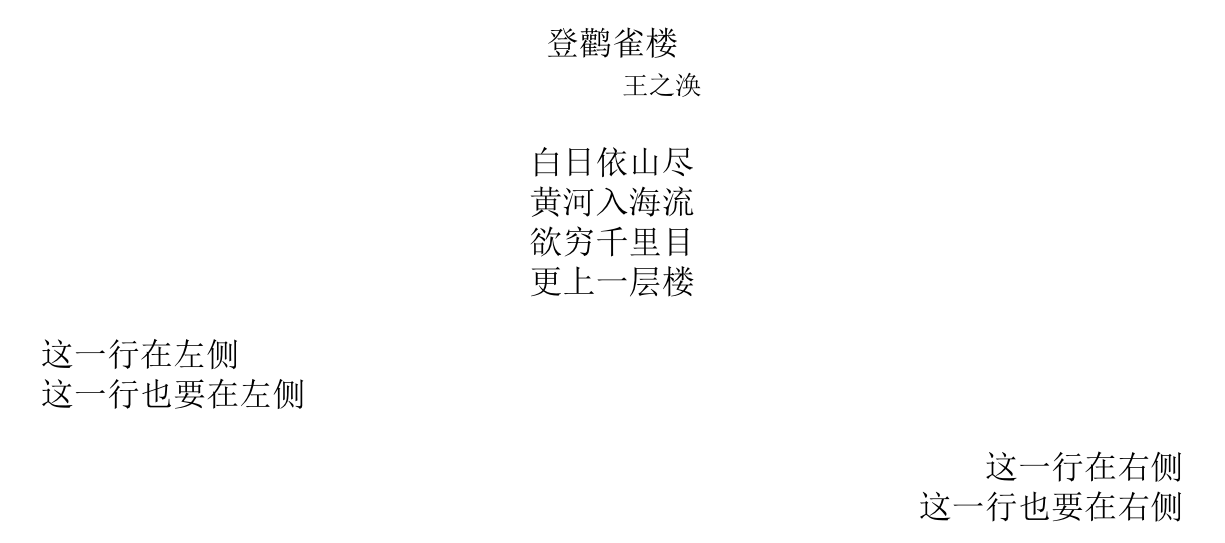verbatim 居中显示
verbatim环境(抄录环境)使LaTex源文件的内容原样呈现于最终文档。这些内容不受center, flushleft, flushright等命令的影响。若想让verbatim环境中内容居中显示，需要使用 verbatimbox等扩展包.
首先在导言区加入:
\usepackage{verbatimbox}

用verbbox环境界定显示内容，然后可通过\theverbbox引用这个verbbox环境对象。
\begin{verbbox}
#include <stdio.h>

void main()
{
printf("Hello World! \n");
}
\end{verbbox}

\begin{figure}[ht]
\centering            %% 居中显示
\theverbbox
\end{figure}

同理，也可以使用flushleft, flushright 等命令实现左对齐和右对齐等效果。
线框
使用\fbox给文字加线框; 使用\parbox给段落添加线框.
\fbox{A}

\hfill

\fbox{
Use $\backslash fbox$ environment for creating line box.
}

\begin{center}
\fbox{
Box lies in center. However
}
\end{center}

\begin{center}
\fbox{
\parbox{\textwidth}{
\begin{center}
This is a \\
Full size box  \\
\end{center}
}
}
\end{center}

\begin{center}
\fbox{
\parbox{0.4\textwidth}{
\begin{center}
This is a \\
Small size box  \\
\end{center}
}
}
\end{center}

显示效果如图所示：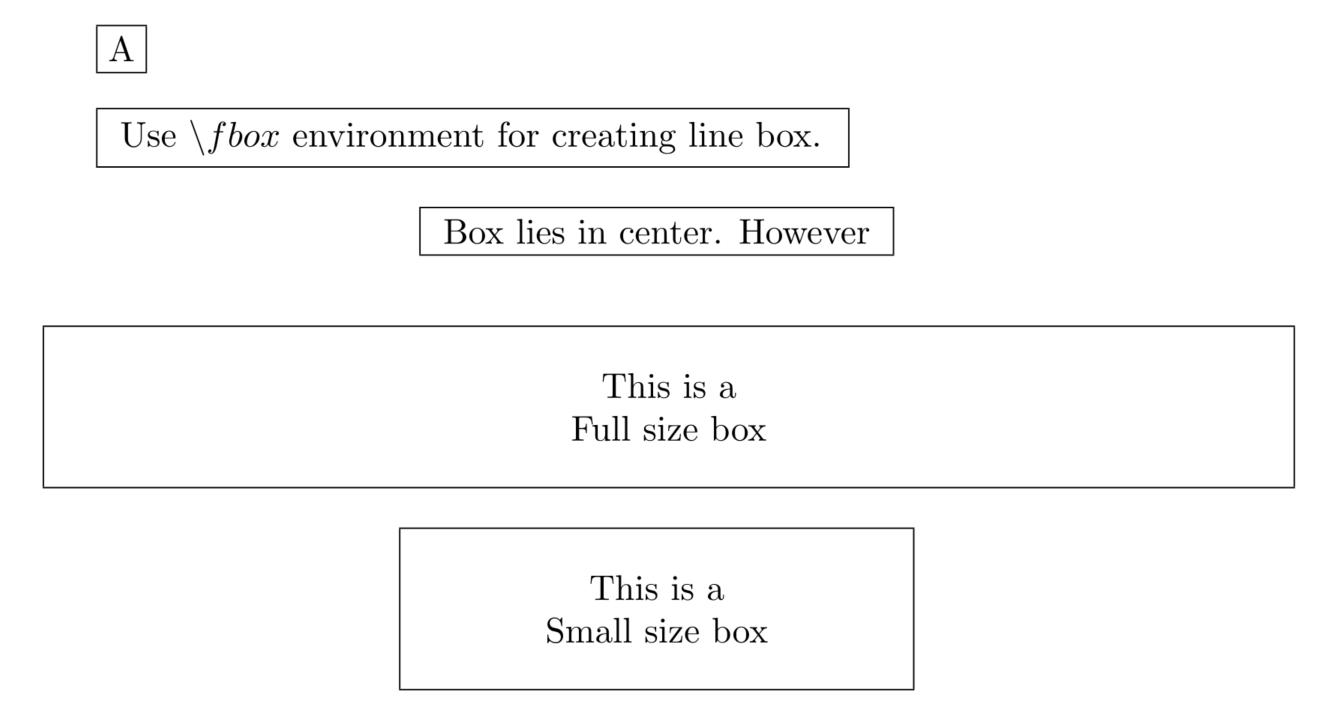图片插入及引用
下面样例展示如何在文档中插入图片并在文中通过图片编号引用图片。
\usepackage[pdftex]{graphicx}
% 设置图片文件存放路径
\graphicspath{{../figures}

\begin{document}
% 在正文中引用图片时使用\ref
In Figure \ref{fig:foo}
\begin{figure}
%设置对齐格式
\centering   %图片居于页面中部
%指定图形大小和图形名称
\includegraphics [width=0.8，height=2.5]{foo.png}
%设置标题
\caption{Foo}
%设置图形引用名称
\label{fig:foo}
\end{figure}

\end{document}

有关插入图形的更多设置请参阅
表格
Latex中使用tabular环境定义表格,  例如
\begin{tabular}{llr}
\hline
\multicolumn{2}{c}{Item} \\
\cline{1-2}
Animal    & Description & Price (\) \\ \hline Gnat & per gram & 13.65 \\ & each & 0.01 \\ Gnu & stuffed & 92.50 \\ Emu & stuffed & 33.33 \\ Armadillo & frozen & 8.99 \\ \hline \end{tabular}  表格对齐格式 首先要在第一行设置表格中各个单元对齐格式。 \begin{tabular}[pos]{table spec}  table spec选项 选项 释意 样例 l 左对齐 {l c r} c 居中 {l c r} r 右对齐 {l c r} p{‘width’} 置顶 {l c p{5cm}} m{‘width’} 纵向居中 {l c m{5cm}} b{‘width’} 置底 {l c b{5cm}} | 纵向分隔线 {l | c |r } || 纵向双分割线 {|| l | c |r ||} 在table spec选项中可使用 >{\format} 设置字体 pos 选项通常用于设置表格单元中显示内容相对于其基线的纵向位置.一般不使用这个选项. 从第二行开始设置表格每一行中列向单元的数量，对齐格式等相关配置. 选项 释意 & 列分隔 \ 新列 \hline 画一条水平线 \newline 在列中换行 \cline{i-j} 在水平方向从第 i 列 到 第 j 列画横线段 表格中行间距 \arraystretch 命令可以设置行间距(默认值是1.0)，例如 \renewcommand{\arraystretch}{1.5}  使用\noalign{\smallskip} 也可以改变行间距. \smallskip 命令等价于\vspace{smallskipamount}。smallskipamount的大小依赖于文档类型. 与\smallskip 命令类似的还有\medskip,\bigskip . 另外，也可以在每一行尾的 换行符 后设置行间距。 例如 \begin{tabular}{c} \hline Normal \\ \hline smallskip(under the characters) \\ \noalign{\smallskip} \hline \noalign{\smallskip} smallskip(over the characters) \\ \hline \noalign{\medskip} medskip \\ \hline \noalign{\bigskip} bigskip \\ \hline 0.5cm \\[0.5cm] \hline \end{tabular}  效果如图所示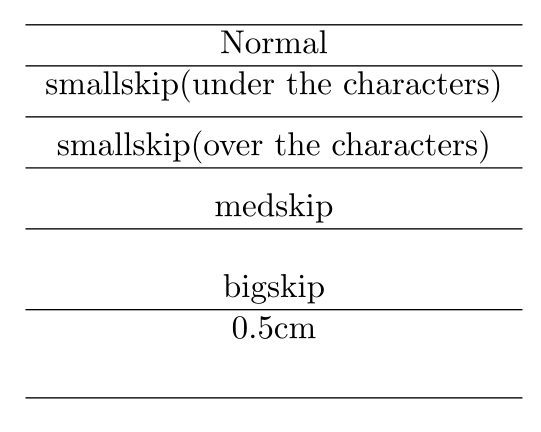三线表样例 Latex中默认的线条宽度是0.4pt, 如果想要使用粗一点的线条，可以使用 booktabs环境包. 这需要在Latex文档的导言部分添加命令: \usepackage{booktabs}  样例如下所示 \begin{table}[h] \centering \begin{tabular}{ccc} \toprule Name & ID & Gender\\ \midrule Tom & 001& Male\\ Rose & 002& Female\\ \bottomrule \end{tabular} \caption{这是一张三线表} \end{table}  其中\toprule 命令是画出表格最上边的一条粗实线(rule). \bottomrule命令是画出表格最下边的一条粗实线. \midrule 命令是画出表格中间的细实线。 效果如下图所示：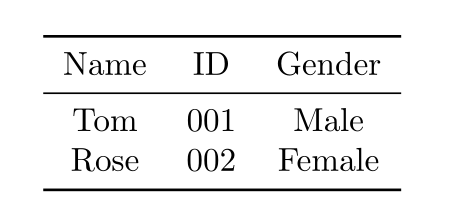有关表格的更多设置请参阅 浮动体 文档中通常需要插入图片或表格以辅助正文表述。图片和表格的内容丰富，一般在一个页面中纵跨多行，并伴有标题等辅助性内容。LaTex将一个图片或表格当作单独整体并定义为浮动体。 为了避免不合理的分页，它们的显示位置不受限于上下文坐标， LaTeX根据相应的算法插入到合适的位置。为了达到理想的排版效果，LaTeX算法可接受位置描述符等参数调配图表位置。位置描述符有以下几种: 描述符 期望位置 h 将浮动体放在这里(Latex会根据其排版美学做调整) Place the float here, i.e., approximately at the same point it occurs in the source text (however, not exactly at the spot) ) t 将浮动体放在页的顶部 Position at the top of the page. b 将浮动体放在页的底部 Position at the bottom of the page. p 将浮动体单独成页 Put on a special page for floats only. ! 忽略LaTeX的排版美学内置参数 Override internal parameters LaTeX uses for determining “good” float positions. H 将浮动体强制放在这里 Places the float at precisely the location in the LaTeX code. Requires the float package,i.e., \usepackage{float}. This is somewhat equivalent to !ht. 画图 Latex中的绘图工具有picture扩展包, tikz扩展包等. 其中picture扩展包是标准扩展包。正文中可直接使用picture包命令绘图，而使用tikz扩展包时则需要在导言中添加 \usepackage{tikz}  ( picture 和 CJK一起使用能正常编译. tikz 和CJK两个扩展包之间有兼容问题. 使用tikz 和CJKutf8的utf8编码文件能正常编译, 但不能在tikz环境中直接中文. 可以通过\mbox{}在tikz环境中插入中文字符 ) 本节主要介绍的picture扩展包和tikz扩展包的基本绘图命令. picture扩展包 picture扩展包中命令主要分为两类: 绘图环境设置命令和绘图命令。 绘制的基本图形有: 直线段，带箭头直线段，二阶贝塞尔曲线段，圆及椭圆等. 下面是一个picture扩展包使用样例 \setlength{\unitlength}{1cm} \thicklines \begin{picture}(10,6) \put(2,2.2){\line(1,0){6}} \put(2,2.2){\circle{2}} \put(6,2.2){\oval(4,2)[r]} \end{picture}  绘图环境设置命令 \begin{picture}(width,height)(x-offset,y-offset)  picture扩展包默认所绘制图形的坐标范围是二维矩形. width,height分别设定图形绘制范围的宽度和高度， 度量单位由 \setlength{\unitlength}{1cm}  设定 (\setlength不是 picture扩展包的命令). \unitlength 默认单位是 1pt. x-offset和y-offset是\begin{picture}命令的可选项, 它们分别设定左下角点的横向坐标值和纵向坐标值 picture中基本绘图命令有 \put(x, y){object} \qbezier(x1, y1)(x2, y2)(x3, y3) \multiput(x, y)(dx, dy){n}{object}  基本图形有： 线段 \put(x, y){ \line(x1, y1){length} }  {length} – 线段在水平方向投影长度(如果垂直线段，则是垂直方向投影长度) (x1, y1) – 线段的方向向量. 方向向量中元素仅限于在(−6, −5, … , 5, 6) 中取值. x1 与 y1要互质. 因此，方向向量总计只有25个，如下所示: \setlength{\unitlength}{5cm} \begin{picture}(1,1) \put(0,0){\line(0,1){1}} \put(0,0){\line(1,0){1}} \put(0,0){\line(1,1){1}} \put(0,0){\line(1,2){.5}} \put(0,0){\line(1,3){.3333}} \put(0,0){\line(1,4){.25}} \put(0,0){\line(1,5){.2}} \put(0,0){\line(1,6){.1667}} \put(0,0){\line(2,1){1}} \put(0,0){\line(2,3){.6667}} \put(0,0){\line(2,5){.4}} \put(0,0){\line(3,1){1}} \put(0,0){\line(3,2){1}} \put(0,0){\line(3,4){.75}} \put(0,0){\line(3,5){.6}} \put(0,0){\line(4,1){1}} \put(0,0){\line(4,3){1}} \put(0,0){\line(4,5){.8}} \put(0,0){\line(5,1){1}} \put(0,0){\line(5,2){1}} \put(0,0){\line(5,3){1}} \put(0,0){\line(5,4){1}} \put(0,0){\line(5,6){.8333}} \put(0,0){\line(6,1){1}} \put(0,0){\line(6,5){1}} \end{picture}  带箭头线段 \put(x, y){\vector(x1, y1){length}}  (x1, y1) – 线段的方向向量. 方向向量中元素仅限于在(−4, −3, … , 3, 4) 中取值. x1 与 y1要互质.如下所示: \setlength{\unitlength}{0.75mm} \begin{picture}(60,40) \put(30,20){\vector(1,0){30}} \put(30,20){\vector(4,1){20}} \put(30,20){\vector(3,1){25}} \put(30,20){\vector(2,1){30}} \put(30,20){\vector(1,2){10}} \thicklines \put(30,20){\vector(-4,1){30}} \put(30,20){\vector(-1,4){5}} \thinlines \put(30,20){\vector(-1,-1){5}} \put(30,20){\vector(-1,-4){5}} \end{picture}  圆形 \put(x, y){\circle{diameter}}  (x, y) – 圆心坐标 {diameter} – 直径，circle命令画出圆的最大直径约为14mm \circle* – 画实心圆 字符 \put(0.7,0.3){A$}  例如: \setlength{\unitlength}{0.8cm} \begin{picture}(6,5) \thicklines \put(1,0.5){\line(2,1){3}} \put(4,2){\line(-2,1){2}} \put(2,3){\line(-2,-5){1}} \put(0.7,0.3){$A$} \put(4.05,1.9){$B$} \put(1.7,2.95){$C$} \put(3.1,2.5){$a$} \put(1.3,1.7){$b$} \put(2.5,1.05){$c$} \put(0.3,4){$F=\sqrt{s(s-a)(s-b)(s-c)}$} \put(3.5,0.4){$\displaystyle s:=\frac{a+b+c}{2}$} \end{picture}  椭圆 \put(x, y){\oval(w, h)}  \put(x, y){\oval(w, h)[position]}  二阶贝塞尔曲线 \qbezier(x1, y1)(x, y)(x2, y2)  \multiput(x, y)(dx, dy ){n}{object}  例如  \linethickness{0.075mm} \multiput(0,0)(1,0){26} {\line(0,1){20}}  用户自定义图形 用户可使用 \newsavebox, \savebox 和\usebox命令设置自定义图形。一个savebox是一类未修饰的盒子(box)。\newsavebox命令声明一个盒子。\savebox命令设定盒子(box)的内容。 \usebox命令将盒子插入文档中。 (A savebox is an non-rendered box that is saved for later repeated rendering via the usebox command. The command \newsavebox creates a placeholder for storing content; the command \savebox stores the specified content in the placeholder without rendering it in the document; and \usebox renders the content of the placeholder into the document.) 例如: \newsavebox{foo}  声明 一个名为foo的盒子. \savebox{foo}(width,height)[position]{content}  定义 盒子foo \put(x, y){\usebox{foo}}  调用盒子foo 例如: \newsavebox{\foldera} \savebox{\foldera} (40,32)[bl]{% definition \multiput(0,0)(0,28){2} {\line(1,0){40}} \multiput(0,0)(40,0){2} {\line(0,1){28}} \put(1,28){\oval(2,2)[tl]} \put(1,29){\line(1,0){5}} \put(9,29){\oval(6,6)[tl]} \put(9,32){\line(1,0){8}} \put(17,29){\oval(6,6)[tr]} \put(20,29){\line(1,0){19}} \put(39,28){\oval(2,2)[tr]} } \newsavebox{\folderb} \savebox{\folderb} (40,32)[l]{% definition \put(0,14){\line(1,0){8}} \put(8,0){\usebox{\foldera}} } \setlength{\unitlength}{0.5mm} \begin{picture}(120,168) \put(34,26){\line(0,1){102}} \put(14,128){\usebox{\foldera}} \multiput(34,86)(0,-37){3} {\usebox{\folderb}} \end{picture}  savebox与 {picture}环境没有依赖关系。将savebox的定义放在 {picture}环境之外，那么整个文档都可以使用这个savebox。 样例 下面是几个使用picture环境画图的样例 \setlength{\unitlength}{1mm} \begin{picture}(120,68) \put(0,28){\vector(1,0){115}} \put(116,27){$x_A$} \put(53,7){\vector(0,1){61}} \put(46,66){$ct_A$} \put(2,27){\line(3,1){110}} \multiput(53,28)(-12,12){2}{\circle*{2}} \put(77,52){\circle*{2}} \put(53,28){\vector(-1,1){11}} \put(53,28){\vector(1,1){23}} \put(54,23){$E_1$} \put(35,41){$E_2$} \put(71,53){$E_3$} \put(28,2){$B'$} \multiput(30,7)(2,6){9}{\line(1,3){1.5}} \put(44,2){$B$} \put(46,7){\line(1,3){17.5}} \put(51,2){$A$} \put(60,2){$B''$} \multiput(62,7)(2,6){9}{\line(1,3){1.5}} \put(83,54){\line(1,-1){5}} \put(89,48){Gerade der} \put(89,44){Gleichzeitigkeit} \put(15,54){$\alpha=\alpha'$} \put(15,52){\line(1,0){10}} % draw_arc P1=(54/43) P2=(53/40) P3=(53/41) r=25 \qbezier(60.906,51.717)(57.057,53.000)(53.000,53.000) % draw_arc P1=(54/43) P2=(53/40) P3=(53/41) r=26 \qbezier(61.222,52.666)(57.219,54.000)(53.000,54.000) % \put(57.219,66.000){\makebox(0,0){$\alpha$}} \put(58.000,56.500){\makebox(0,0){$\alpha$}} % draw_arc P1=(6/40) P2=(5/40) P3=(8/41) r=20 \qbezier(25.000,28.000)(25.000,31.246)(23.974,34.325) % draw_arc P1=(6/40) P2=(5/40) P3=(8/41) r=21 \qbezier(26.000,28.000)(26.000,31.408)(24.922,34.641) \put(29.000,32.408){\makebox(0,0){$\alpha'$}} \end{picture}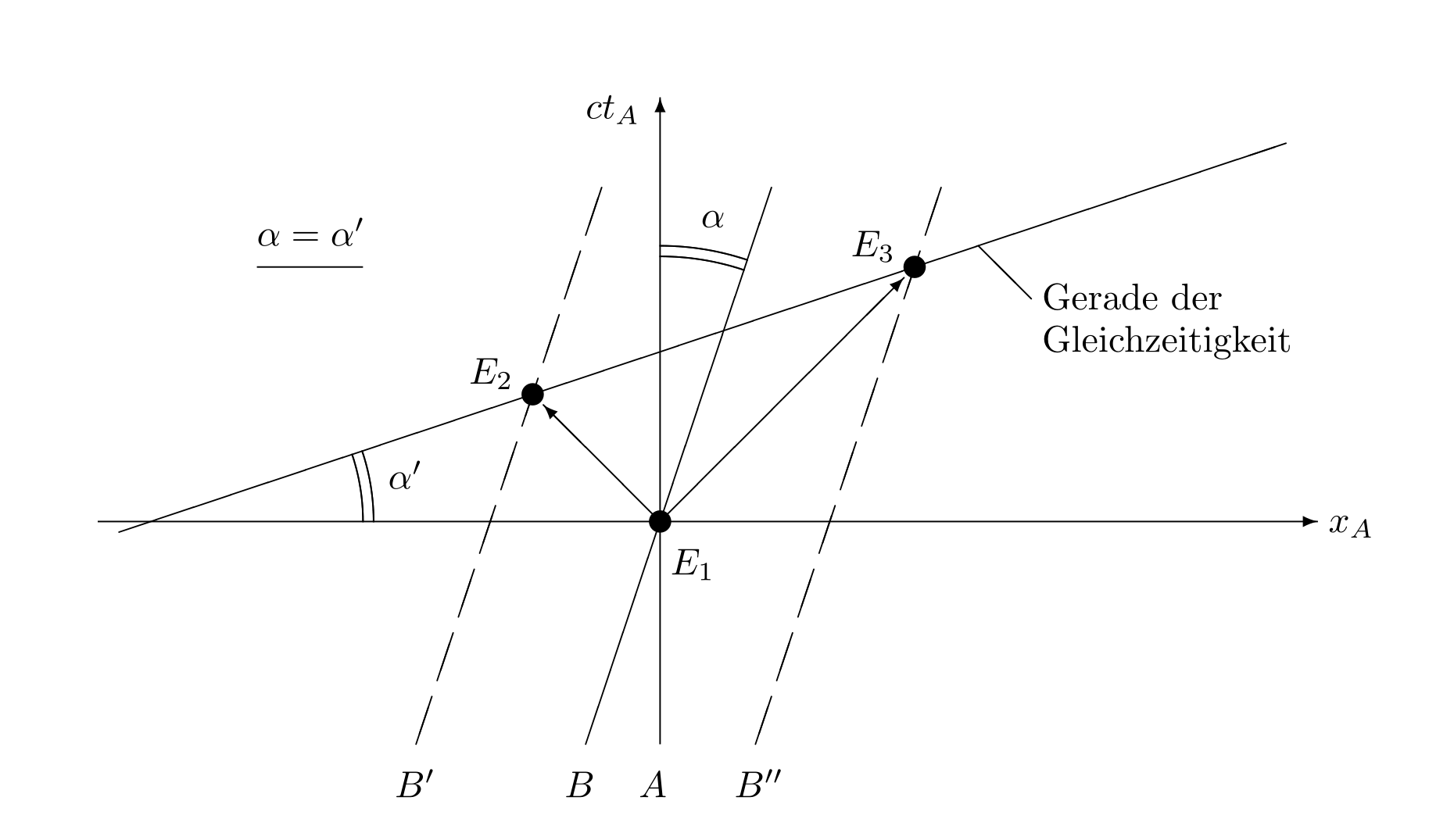\setlength{\unitlength}{1mm} \begin{picture}(96,38) \put(0,12){\vector(1,0){91}} \put(92,11){$x_L$} \put(6,10){$\underbrace{\rule{4cm}{0cm}}$} \put(26,5){\makebox(0,0){$v\cdot\Delta t_L$}} \multiput(1,12)(0,20){2}{\line(1,0){10}} \multiput(1,12)(10,0){2}{\line(0,1){20}} \multiput(41,12)(0,20){2}{\line(1,0){10}} \multiput(41,12)(10,0){2}{\line(0,1){20}} \multiput(6,12)(40,0){2}{\circle*{2}} \put(46,32){\circle*{2}} \put(46,12){\line(0,1){20}} \put(6,12){\vector(2,1){39}} \put(18,25){$c\cdot\Delta t_L$} \put(46,32){\vector(2,-1){39}} \put(46,22){\line(2,-1){8}} \put(54.5,16){$h=c\cdot\Delta\tau$} \end{picture}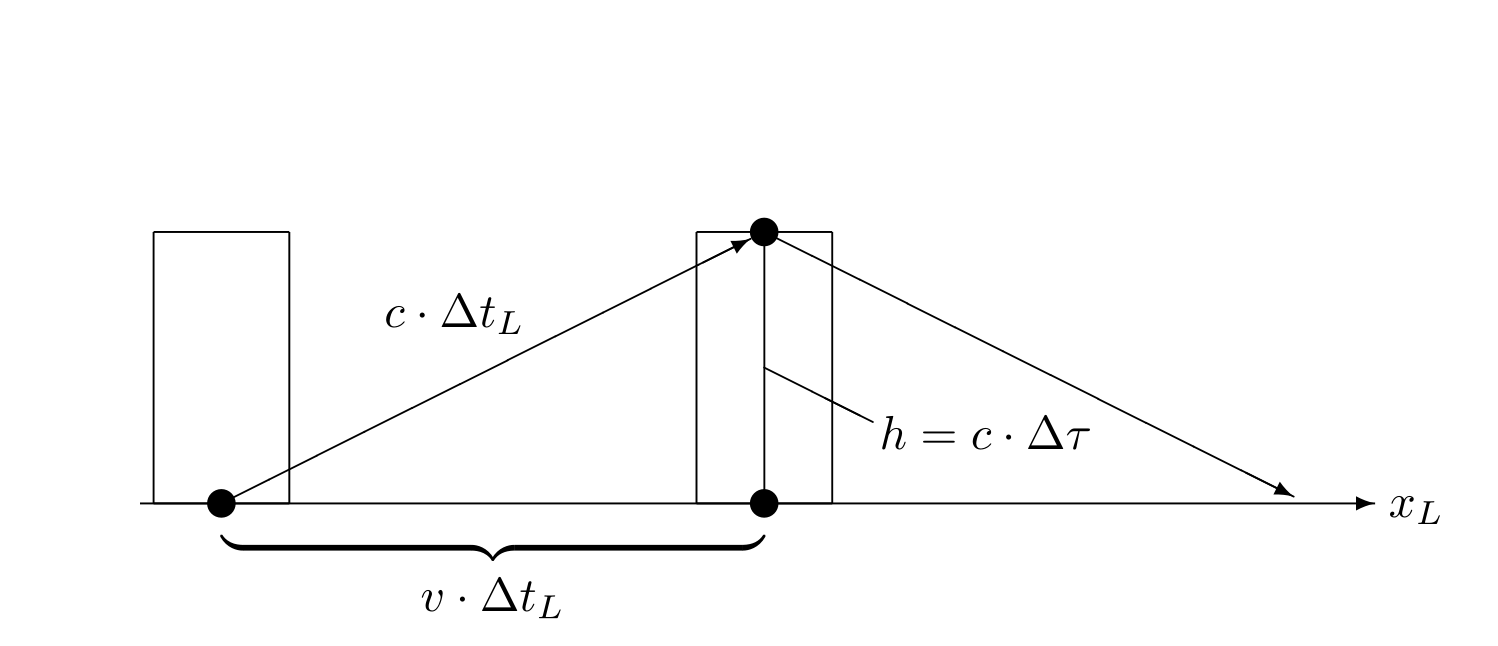\setlength{\unitlength}{1mm} \begin{picture}(93,46) \put( 0,14){\vector(1,0){60}} \put(61,13){$x$} \put(20,4){\vector(0,1){37}} \put(19,43){$y$} \put(50,34){\circle*{2}} \put(52,35){$P$} \multiput(20,34)(4,0){8}{\line(1,0){2}} \put(14.5,33.5){$y_P$} \multiput(50,14)(0,4){5}{\line(0,1){2}} \put(48,11){$x_P$} \put( 2,8){\vector(3,1){56}} \put(59,26.5){$x'$} \multiput(50,34)(1.9,-5.7){2} {\line(1,-3){1.2}} \put(52,22){$x_P'$} \multiput(50,34)(-5.8,-1.933){6} {\line(-3,-1){3.6}} \put(12,21){$y_P'$} \put(22,8){\vector(-1,3){10.5}} \put(10,41){$y'$} \end{picture}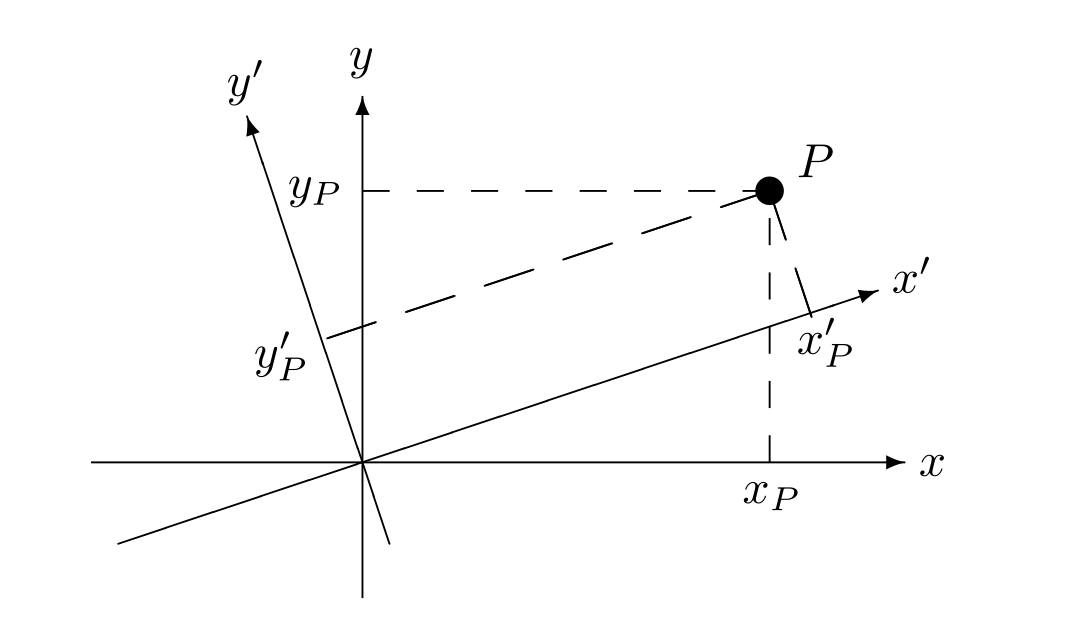tikz包 使用tikz包绘制基本图形样例 绘图环境的坐标系 tikz环境中绘制的是二维图形， 在设定点坐标时，既可以使用卡氏坐标(Cartesian coordinates)，也就是我们常用的直角坐标系，例如 (1cm, 10pt)  也可以使用极坐标(polar coordinates) (30:1cm)  中文字符 在tikz环境中使用中文，可以用CJKutf8环境，tex文件保存格式为utf8, 通过\mbox{}在tikz环境中插入中文字符. 例如: \path (0,4.6) node[left] {\mbox{中文}}  直线段 \draw (0,0) -- (4,0);  多个相连的直线段 \draw (0,0) -- (4,0) -- (4,4) -- (0,4) -- (0,0);  封闭的直线段环 \draw (0,0) -- (4,0) -- (4,4) -- (0,4) -- cycle;  贝塞尔曲线 \draw (0,0) .. controls (0,4) and (4,0) .. (4,4);  抛物线 \draw (0,0) parabola (4,4);  圆弧 \draw (3,0) arc (0:75:3cm);  圆 \draw (2,2) circle (3cm);  椭圆 \draw (2,2) ellipse (3cm and 1cm);  矩形 \draw (0,0) rectangle (4,4);  设置线型 \draw[red,thick,dashed] (2,2) circle (3cm);  网格 \draw[step=1cm,gray,very thin] (-2,-2) grid (6,6);  无边界网格(缩小绘图范围，达到消隐网格边界的效果) \draw[step=1cm,gray,very thin] (-1.9,-1.9) grid (5.9,5.9);  坐标轴 \draw[thick,->] (0,0) -- (4.5,0); \draw[thick,->] (0,0) -- (0,4.5);  端点带标注坐标轴 \draw[thick,->] (0,0) -- (4.5,0) node[anchor=north west] {x axis}; \draw[thick,->] (0,0) -- (0,4.5) node[anchor=south east] {y axis};  坐标轴刻度 \foreach \x in {0,1,2,3,4} \draw (\x cm,1pt) -- (\x cm,-1pt) node[anchor=north] {$\x$}; \foreach \y in {0,1,2,3,4} \draw (1pt,\y cm) -- (-1pt,\y cm) node[anchor=east] {$\y$};  Kjell Magne Fauske提供的使用tikz包画图样例: \documentclass[UTF8]{article} \usepackage{tikz} \begin{document} \newpage \pagestyle{empty} % 3D Cone % Author: Gene Ressler. Adapted to TikZ by Kjell Magne Fauske. % See http://www.frontiernet.net/~eugene.ressler/ for more details. % The following code is generated by Sketch. I have edited it a bit % to make it easier to read. \begin{tikzpicture} \tikzstyle{conefill} = [fill=blue!20,fill opacity=0.8] \tikzstyle{ann} = [fill=white,font=\footnotesize,inner sep=1pt] \tikzstyle{ghostfill} = [fill=white] \tikzstyle{ghostdraw} = [draw=black!50] \filldraw[conefill](-.775,1.922)--(-1.162,.283)--(-.274,.5) --(-.183,2.067)--cycle; \filldraw[conefill](-.183,2.067)--(-.274,.5)--(.775,.424) --(.516,2.016)--cycle; \filldraw[conefill](.516,2.016)--(.775,.424)--(1.369,.1) --(.913,1.8)--cycle; \filldraw[conefill](-.913,1.667)--(-1.369,-.1)--(-1.162,.283) --(-.775,1.922)--cycle; \draw(1.461,.107)--(1.734,.127); \draw[arrows=<->](1.643,1.853)--(1.643,.12); \filldraw[conefill](.913,1.8)--(1.369,.1)--(1.162,-.283) --(.775,1.545)--cycle; \draw[arrows=->,line width=.4pt](.274,-.5)--(0,0)--(0,2.86); \draw[arrows=-,line width=.4pt](0,0)--(-1.369,-.1); \draw[arrows=->,line width=.4pt](-1.369,-.1)--(-2.1,-.153); \filldraw[conefill](-.516,1.45)--(-.775,-.424)--(-1.369,-.1) --(-.913,1.667)--cycle; \draw(-1.369,.073)--(-1.369,2.76); \draw(1.004,1.807)--(1.734,1.86); \filldraw[conefill](.775,1.545)--(1.162,-.283)--(.274,-.5) --(.183,1.4)--cycle; \draw[arrows=<->](0,2.34)--(-.913,2.273); \draw(-.913,1.84)--(-.913,2.447); \draw[arrows=<->](0,2.687)--(-1.369,2.587); \filldraw[conefill](.183,1.4)--(.274,-.5)--(-.775,-.424) --(-.516,1.45)--cycle; \draw[arrows=<-,line width=.4pt](.42,-.767)--(.274,-.5); \node[ann] at (-.456,2.307) {$r_0$}; \node[ann] at (-.685,2.637) {$r_1$}; \node[ann] at (1.643,.987) {$h$}; \path (.42,-.767) node[below] {$x$} (0,2.86) node[above] {$y$} (-2.1,-.153) node[left] {$z$}; % Second version of the cone \begin{scope}[xshift=3.5cm] \filldraw[ghostdraw,ghostfill](-.775,1.922)--(-1.162,.283)--(-.274,.5) --(-.183,2.067)--cycle; \filldraw[ghostdraw,ghostfill](-.183,2.067)--(-.274,.5)--(.775,.424) --(.516,2.016)--cycle; \filldraw[ghostdraw,ghostfill](.516,2.016)--(.775,.424)--(1.369,.1) --(.913,1.8)--cycle; \filldraw[ghostdraw,ghostfill](-.913,1.667)--(-1.369,-.1)--(-1.162,.283) --(-.775,1.922)--cycle; \filldraw[ghostdraw,ghostfill](.913,1.8)--(1.369,.1)--(1.162,-.283) --(.775,1.545)--cycle; \filldraw[ghostdraw,ghostfill](-.516,1.45)--(-.775,-.424)--(-1.369,-.1) --(-.913,1.667)--cycle; \filldraw[ghostdraw,ghostfill](.775,1.545)--(1.162,-.283)--(.274,-.5) --(.183,1.4)--cycle; \filldraw[fill=red,fill opacity=0.5](-.516,1.45)--(-.775,-.424)--(.274,-.5) --(.183,1.4)--cycle; \fill(-.775,-.424) circle (2pt); \fill(.274,-.5) circle (2pt); \fill(-.516,1.45) circle (2pt); \fill(.183,1.4) circle (2pt); \path[font=\footnotesize] (.913,1.8) node[right] {$i\hbox{$=$}0$} (1.369,.1) node[right] {$i\hbox{$=$}1$}; \path[font=\footnotesize] (-.645,.513) node[left] {$j$} (.228,.45) node[right] {$j\hbox{$+$}1$}; \draw (-.209,.482)+(-60:.25) [yscale=1.3,->] arc(-60:240:.25); \fill[black,font=\footnotesize] (-.516,1.45) node [above] {$P_{00}$} (-.775,-.424) node [below] {$P_{10}$} (.183,1.4) node [above] {$P_{01}$} (.274,-.5) node [below] {$P_{11}$}; \end{scope} \end{tikzpicture} \end{document}  效果如下图所示：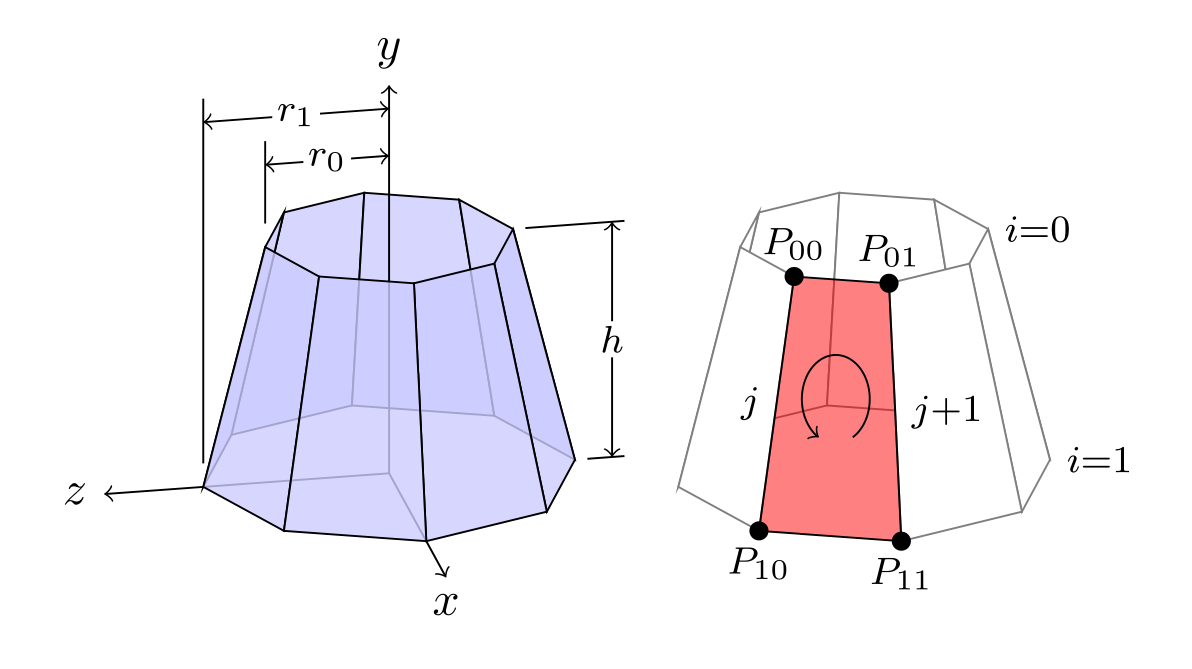动画 使用LaTex在pdf文件中插入动画需要{animate}扩展包，可在LaTex文件引导区添加 \usepackage{animate}  插入动画命令\animategraphics的语法是 \animategraphics[<options>]{<frame rate>}{<file basename>}{<first>}{<last>}  < frame rate>定义动画的帧速，< file basename>图形文件名的基， < first>、< last>图形文件起始和结束的序号。例如:panda0.png, panda1.png, …, panda100.png 附： gif动画分解网址: https://ezgif.com/split/ http://www.webtoolss.com/gif_bunkai.html 样例:  \animategraphics[ autoplay,loop,controls, width=.7\textwidth, height=.7\textheight ]{4}{./pandagif/panda}{0}{100}  其中动画图片panda0.png, panda1.png, …, panda100.png都保存在pandagif文件夹中 时间轴(timeline) 画时间轴 下面这个例子基于table画竖向时间轴. 只使用了xcolor扩展包， \documentclass{article} \usepackage{xcolor} \newcommand\ytl{ \parbox[b]{8em}{\hfill{\color{cyan}\bfseries\sffamily #1}~$\cdots\cdots$~}\makebox[0pt][c]{$\bullet$}\vrule\quad \parbox[c]{4.5cm}{\vspace{7pt}\color{red!40!black!80}\raggedright\sffamily #2.\\[7pt]}\\[-3pt]} \begin{document} \begin{table} \caption{Timeline of something.} \centering \begin{minipage}[t]{.7\linewidth} \color{gray} \rule{\linewidth}{1pt} \ytl{1947}{AT and T Bell Labs develop the idea of cellular phones} \ytl{1968}{Xerox Palo Alto Research Centre envisage the Dynabook'} \ytl{1971}{Busicom 'Handy-LE' Calculator} \ytl{1973}{First mobile handset invented by Martin Cooper} \ytl{1978}{Parker Bros. Merlin Computer Toy} \ytl{1981}{Osborne 1 Portable Computer} \ytl{1982}{Grid Compass 1100 Clamshell Laptop} \ytl{1983}{TRS-80 Model 100 Portable PC} \ytl{1984}{Psion Organiser Handheld Computer} \ytl{1991}{Psion Series 3 Minicomputer} \bigskip \rule{\linewidth}{1pt}% \end{minipage}% \end{table} \end{document}  显示效果如下：流程图 使用tikz宏包画流程图样例如下： \documentclass[UTF8]{article} %% 在导言中设置tikz扩展包 \usepackage{tikz} \usetikzlibrary{arrows, shapes.geometric, decorations.pathmorphing, backgrounds, positioning, fit, petri, automata} \begin{document} \thispagestyle{empty} % 在正文中定义流程图中控件样式 \tikzstyle{startstop} = [rectangle, rounded corners, minimum width=3cm, minimum height=1cm,text centered, draw=black, fill=red!30] \tikzstyle{io} = [trapezium, trapezium left angle=70, trapezium right angle=110, minimum width=3cm, minimum height=1cm, text centered, draw=black, fill=blue!30] \tikzstyle{process} = [rectangle, minimum width=3cm, minimum height=1cm, text centered, draw=black, fill=orange!30] \tikzstyle{decision} = [diamond, minimum width=3cm, minimum height=1cm, text centered, draw=black, fill=green!30] \tikzstyle{Data} = [rectangle, rounded corners, minimum width=3cm, minimum height=1cm,text centered, draw=black, fill=red!30] \tikzstyle{arrow} = [thick,->,>=stealth] %% 设置流程图内容 \begin{tikzpicture}[node distance=2cm] %定义流程图具体控件 \node (start) [startstop] {Start}; \node (in1) [io, below of=start] {Input}; \node (pro1) [process, below of=in1] {Process 1}; \node (dec1) [decision, below of=pro1, yshift=-0.5cm] {Decision 1}; \node (pro2a) [process, below of=dec1, yshift=-0.5cm] {Process 2a}; \node (pro2b) [process, right of=dec1, xshift=2cm] {Process 2b}; \node (out1) [io, below of=pro2a] {Output}; \node (stop) [startstop, below of=out1] {Stop}; %连接控件 \draw [arrow](start) -- (in1); \draw [arrow](in1) -- (pro1); \draw [arrow](pro1) -- (dec1); \draw [arrow](dec1) -- (pro2a); \draw [arrow](dec1) -- (pro2b); \draw [arrow](dec1) -- node[anchor=east] {yes} (pro2a); \draw [arrow](dec1) -- node[anchor=south] {no} (pro2b); \draw [arrow](pro2b) |- (pro1); \draw [arrow](pro2a) -- (out1); \draw [arrow](out1) -- (stop); \end{tikzpicture} \end{document}  数学公式 按照数学公式在文中的位置可分为两种: 行中公式和独立公式. 行中公式 行中公式可使用如下两种形式: 毕达哥拉斯定理 \begin{math} x^{2}+y^{2}=z^{2} \end{math}又称勾股定理。  或 毕达哥拉斯定理$ x^{2}+y^{2}=z^{2} $又称勾股定理。  使用上面两种方法编译后得到的文档是一样的，具体如下图所示：独立公式 独立公式可使用 $$v = v^{1}e_{1} + v^{2}e_{2} + v^{3}e_{3} = v^{i}e_{i}, i = 1,2,3$$ 或 \begin{equation} v = v^{1}e_{1} + v^{2}e_{2} + v^{3}e_{3} = v^{i}e_{i}, i = 1,2,3 \end{equation}  效果如下图所示：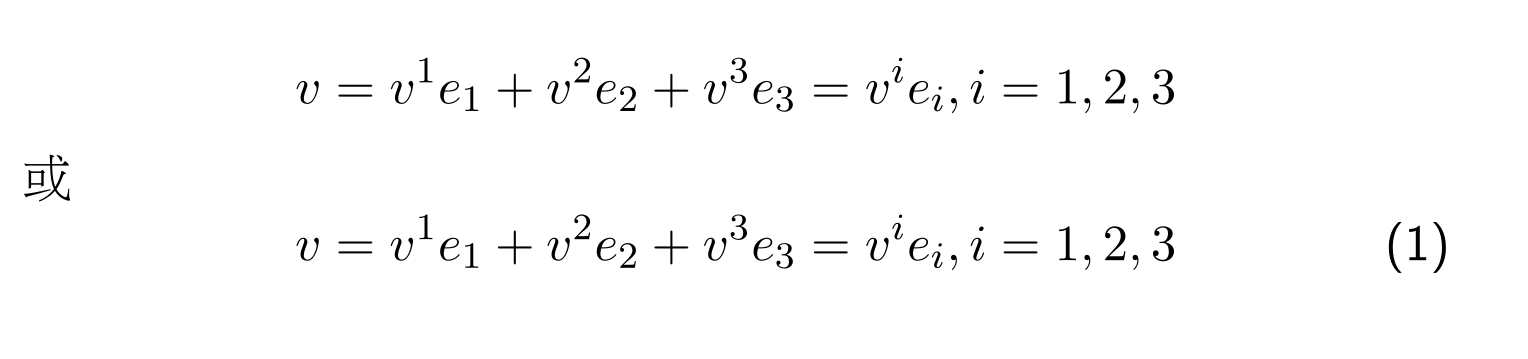由上图可看出，使用 {equation} 命令默认带公式编号，使用 $$命令默认不带公式编号。 数学公式显示样式 有些数学符号会根据文档中上下文的不同而改变其显示形式。例如，在正文环境中和在独立数学公式环境中，分式会有不同的显示样式. f(x) = \sum_{n=0}^{10} \frac{x}{n!} \\$$ f(x) = \sum_{n=0}^{10} \frac{x}{n!} $$如果想改变数学符号的默认显示样式, 可使用下列样式命令设定: \displaystyle \textstyle \scriptscriptstyle 例如： In-line math components can be set with independent math display style f(x) = \displaystyle \sum_{n=0}^{10} \frac{x}{n!}, and vice versa:$$ f(x) = \sum_{n=0}^{10} \frac{x}{n!} $$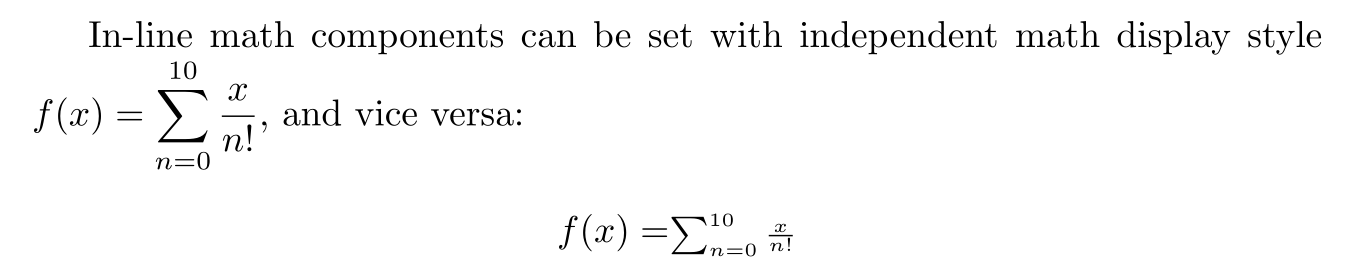引用公式 在文中引用公式编号可使用以下方式 \begin{equation}\label{eq:Pythagorean theorem} x^{2}+y^{2}=z^{2} \end{equation} 公式\ref{eq:Pythagorean theorem}是毕达哥拉斯定理，在中国又称勾股定理。  效果如下：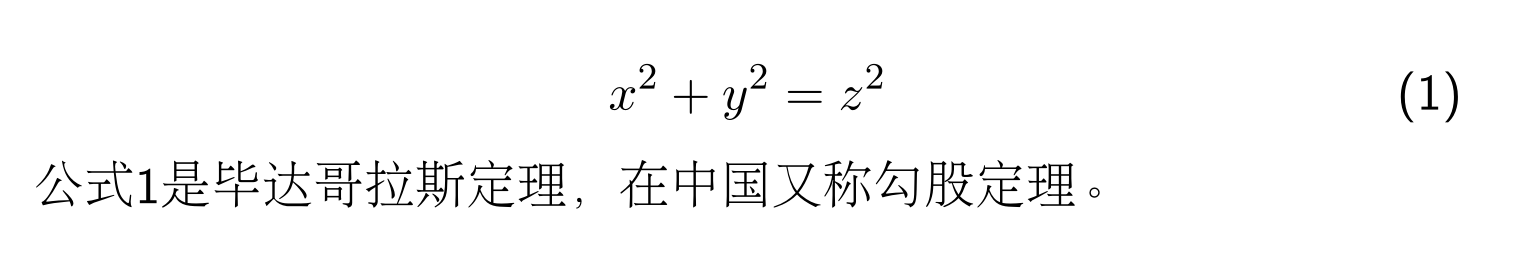在数学公式中插入中文 使用\mbox{}可在数学公式中插入中文。 $$ \mbox{例如：} x_{1}, x_{2}, \cdots, x_{N} $$ 效果如下图：多行公式 $$ \left [ \begin{array}{cc} v^{i} e_{i} & v^{i} e_{j} \\ v^{j} e_{i} & v^{j} e_{j} \\ \end{array} \right ] $$ 效果如下图所示：$$ \left \{ \begin{array}{r c l} (f + g)(x) & = & f(x) + g(x) \\ (\alpha f)(x) & = & \alpha f(x) \\ (fg)(x) & = & f(x)g(x) \\ \end{array} \right . $$ 效果如下图所示：多行公式也可以在{eqnarray}环境实现，例如： \begin{eqnarray} w(0) & = & 0 \\ \frac{\partial w}{\partial x}\Big |_{x=0} & = & 0 \\ \end{eqnarray}  下标 一般数学公式中下标可以使用 $$ s = \int_{a}^{b} |\dot{x}(t)| dt $$ 有时需要将下标放在正下方, 如果是数学符号，可使用 \limits命令 $$ r'(t) = \lim \limits_{\triangle t \rightarrow 0} \frac{ r(t + \triangle t) - r(t)}{ \triangle t }$$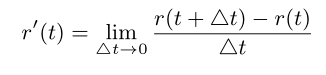如果是普通符号，那么要用KaTeX parse error: Expected group after '\mathop' at end of input: \mathop先转成数学符号再用\limits 长竖线 数学公式中的竖线高度可通过 “\big” “\Big” “\bigg” “\Bigg” 调整。 $$ \frac{\partial w}{\partial x}\Big |_{x=0} = 0$$ 根号 The square root of 100 is$\sqrt{100}=10$. \\ The cubic root of 64 is$\sqrt{64}=4$.  得到的文档如下图所示页脚注释和页边注释 页脚注释 在正文中添加页脚注释的命令是:\footnote, 例如： 正文内容\footnote{注释内容}  Latex会按顺序为脚注设置编号。 也可以使用\footnotemark和\footnotetext 添加页脚注释，例如： 在这里添加页脚注释角标\footnotemark %% ... 在这里设置注释内容\footnotetext{注释内容}  \footnote脚注命令不能在数学公式，表格，子页面等环境中使用。如果想在表格中引用页脚注释，可参考下面的例子: 正文内容\footnote{注释内容\label{fnote}} 在这里也引用与上边脚注相同的角标 \textsuperscript{\ref{fnote}}  页边注释 在正文中添加页边注释的命令是:\marginpar, 例如： \marginpar{注释内容}  关于页脚注释和页边注释命令的使用以及其它内容请参考 枚举项编号样式 使用{enumitem}扩展包 \usepackage{enumitem}  \begin{enumerate}[label=(\alph*)] \item an apple \item a banana \item a carrot \item a durian \end{enumerate} \begin{enumerate}[label=(\Alph*)] \item an apple \item a banana \item a carrot \item a durian \end{enumerate} \begin{enumerate}[label=(\roman*)] \item an apple \item a banana \item a carrot \item a durian \end{enumerate}  使用参考文献 Latex中参考文献命令格式是：  \begin{thebibliography}{9} \bibitem{latexcompanion} Michel Goossens, Frank Mittelbach, and Alexander Samarin. \textit{The \LaTeX\ Companion}. Addison-Wesley, Reading, Massachusetts, 1993. \bibitem{einstein} Albert Einstein. \textit{Zur Elektrodynamik bewegter K{\"o}rper}. (German) [\textit{On the electrodynamics of moving bodies}]. Annalen der Physik, 322(10):891–921, 1905. \bibitem{knuthwebsite} Knuth: Computers and Typesetting, \\\texttt{http://www-cs-faculty.stanford.edu/\~{}uno/abcde.html} \end{thebibliography}  注意：\bibitem命令中 参考文献引用名中可以有空格。 Bibtex是常用的参考文献管理工具。Bibtex将参考文献条目内容保存在一个.bib后缀文件中。 Bibtex能根据参考文献在正文中被引用的顺序自动过滤并调整文献编号，因此不同论文中可以使用同一个bib文件。 Bibtex使用的参考文献格式如下所示： @article{name1, author = {Auther 1 and Auther 2}, title = {The \LaTeX\ Companion}, journal = {Journal name}, volume = {49}, pages = {409-436}, year = {19xx}, abstract = {摘要主要是给自己参考, 这一项不是必须的} } @book{name2, author = {Auther 1 and Auther 2}, title = {The TeX Book}, publisher = "Springer", year = {19xx}, abstract = {摘要} } @article{einstein, author = "Albert Einstein", title = "{Zur Elektrodynamik bewegter K{\"o}rper}. ({German}) [{On} the electrodynamics of moving bodies]", journal = "Annalen der Physik", volume = "322", number = "10", pages = "891--921", year = "1905", DOI = "http://dx.doi.org/10.1002/andp.19053221004" } @book{latexcompanion, author = "Michel Goossens and Frank Mittelbach and Alexander Samarin", title = "The \LaTeX\ Companion", year = "1993", publisher = "Addison-Wesley", address = "Reading, Massachusetts" } @misc{knuthwebsite, author = "Donald Knuth", title = "Knuth: Computers and Typesetting", url = "http://www-cs-faculty.stanford.edu/\~{}uno/abcde.html" } @article{article, author = {Peter Adams}, title = {The title of the work}, journal = {The name of the journal}, year = 1993, number = 2, pages = {201-213}, month = 7, note = {An optional note}, volume = 4 } @book{book, author = {Peter Babington}, title = {The title of the work}, publisher = {The name of the publisher}, year = 1993, volume = 4, series = 10, address = {The address}, edition = 3, month = 7, note = {An optional note}, isbn = {3257227892} } @booklet{booklet, title = {The title of the work}, author = {Peter Caxton}, howpublished = {How it was published}, address = {The address of the publisher}, month = 7, year = 1993, note = {An optional note} } @conference{conference, author = {Peter Draper}, title = {The title of the work}, booktitle = {The title of the book}, year = 1993, editor = {The editor}, volume = 4, series = 5, pages = 213, address = {The address of the publisher}, month = 7, organization = {The organization}, publisher = {The publisher}, note = {An optional note} } @inbook{inbook, author = {Peter Eston}, title = {The title of the work}, chapter = 8, pages = {201-213}, publisher = {The name of the publisher}, year = 1993, volume = 4, series = 5, address = {The address of the publisher}, edition = 3, month = 7, note = {An optional note} } @incollection{incollection, author = {Peter Farindon}, title = {The title of the work}, booktitle = {The title of the book}, publisher = {The name of the publisher}, year = 1993, editor = {The editor}, volume = 4, series = 5, chapter = 8, pages = {201-213}, address = {The address of the publisher}, edition = 3, month = 7, note = {An optional note} } @manual{manual, title = {The title of the work}, author = {Peter Gainsford}, organization = {The organization}, address = {The address of the publisher}, edition = 3, month = 7, year = 1993, note = {An optional note} } @mastersthesis{mastersthesis, author = {Peter Harwood}, title = {The title of the work}, school = {The school of the thesis}, year = 1993, address = {The address of the publisher}, month = 7, note = {An optional note} } @misc{misc, author = {Peter Isley}, title = {The title of the work}, howpublished = {How it was published}, month = 7, year = 1993, note = {An optional note} } @phdthesis{phdthesis, author = {Peter Joslin}, title = {The title of the work}, school = {The school of the thesis}, year = 1993, address = {The address of the publisher}, month = 7, note = {An optional note} } @proceedings{proceedings, title = {The title of the work}, year = 1993, editor = {Peter Kidwelly}, volume = 4, series = 5, address = {The address of the publisher}, month = 7, organization = {The organization}, publisher = {The name of the publisher}, note = {An optional note} } @techreport{techreport, author = {Peter Lambert}, title = {The title of the work}, institution = {The institution that published}, year = 1993, number = 2, address = {The address of the publisher}, month = 7, note = {An optional note} } @unpublished{unpublished, author = {Peter Marcheford}, title = {The title of the work}, note = {An optional note}, month = 7, year = 1993 }  可将上述内容保存到一个.bib后缀文件，例如foo.bib。 注意：bib文件中 参考文献引用名中不能有空格。 在preamble中添加(不加这个命令也能用) \usepackage{cite}  在正文中引用时方法如下： \begin{document} \cite{name1}"The geometric definition of dot product is coordinate independent, ..."\cite{latexcompanion,knuthwebsite} \medskip %% IEEEtran是参考文献显示格式 \bibliographystyle{IEEEtran} %% foo is the bib file name \bibliography{foo} \end{document}  注意： 1) 应用多个文献时，例如：\cite{latexcompanion,knuthwebsite}， 参考文献引用名之间用逗号分隔，不能插入空格。 2) \bibliography{foo} 中使用文件名(本例中是foo)，不能带.bib后缀. 常用的bibliographystyle有: abbrv, acm, alpha, apalike, ieetr, plain,siam, unsrt等。 BibTeX常用的项有: address annote author booktitle chapter crossref edition editor institution journal key month note number organization pages publisher school series title type volume year URL ISBN ISSN LCCN abstract keywords price copyright language contents Beamer样例 %~~~~~~~~~~~~~~~~~~~~~~~~~~~~~~~~~~~~~~~~~~~~~~ % 设置文档类型为 beamer %~~~~~~~~~~~~~~~~~~~~~~~~~~~~~~~~~~~~~~~~~~~~~~ \documentclass{beamer} \usepackage{beamerthemesplit} \usepackage{animate} \title{Example Presentation Created with the Beamer Package} \author{Till Tantau} \date{\today} \begin{document} %~~~~~~~~~~~~~~~~~~~~~~~~~~~~~~~~~~~~~~~~~~~~~~ % TITLE PAGE %~~~~~~~~~~~~~~~~~~~~~~~~~~~~~~~~~~~~~~~~~~~~~~ \frame{\titlepage} \section*{Outline} \frame{\tableofcontents} \section{Introduction} \subsection{Overview of the Beamer Class} %~~~~~~~~~~~~~~~~~~~~~~~~~~~~~~~~~~~~~~~~~~~~~~ % 使用 frame 命令生成一页 %~~~~~~~~~~~~~~~~~~~~~~~~~~~~~~~~~~~~~~~~~~~~~~ \frame { \frametitle{Features of the Beamer Class} \begin{itemize} \item<1-> Normal LaTeX class. \item<2-> Easy overlays. \item<3-> No external programs needed. \end{itemize} } \begin{frame}{Metropolis title formats} supports 4 different title formats: \begin{itemize} \item Regular \item \textsc{Small caps} \item \textsc{all small caps} \item ALL CAPS \end{itemize} They can either be set at once for every title type or individually. \end{frame} %~~~~~~~~~~~~~~~~~~~~~~~~~~~~~~~~~~~~~~~~~~~~~ % 公式于图片并列 %~~~~~~~~~~~~~~~~~~~~~~~~~~~~~~~~~~~~~~~~~~~~~ \begin{frame}{Euler–Bernoulli 梁理论} \begin{figure}[H] \begin{minipage}{0.48\linewidth} \centerline{\includegraphics[width=4.0cm]{foo.PNG}} \end{minipage} \hfill \begin{minipage}{.48\linewidth}$ \displaystyle  \frac{d^{2}}{dx^{2}} (EI_{y} \frac{d^{2} \omega}{dx^{2}}) = q$\end{minipage} \caption{Euler–Bernoulli 方程} \label{zrotate} \end{figure} \end{frame} %~~~~~~~~~~~~~~~~~~~~~~~~~~~~~~~~~~~~~~~~~~~~~ % 插入代码片段， 需要使用 fragile %~~~~~~~~~~~~~~~~~~~~~~~~~~~~~~~~~~~~~~~~~~~~~ \begin{frame} [fragile] \frametitle{Code} \begin{verbatim} int main (int argc, char* argv[]) { printf("Hello world. \n"); return 0; } \end{verbatim} \end{frame} %~~~~~~~~~~~~~~~~~~~~~~~~~~~~~~~~~~~~~~~~~~~~~ % 插入动画 %~~~~~~~~~~~~~~~~~~~~~~~~~~~~~~~~~~~~~~~~~~~~~ \begin{frame} \animategraphics[ autoplay,loop,controls, width=.7\textwidth, height=.7\textheight ]{4}{/pandagif/panda}{0}{100} \end{frame} \end{document}  字体与字号 Latex的字体由小到大分别为 \tiny \scriptsize \footnotesize \small \normalsize \large \Large \LARGE \huge \Huge  例如 如果在文档局部微调某些字句的字体大小：  {\tiny Hello}\\ {\scriptsize Hello}\\ {\footnotesize Hello}\\ {\small Hello}\\ {\normalsize Hello}\\ {\large Hello}\\ {\Large Hello}\\ {\LARGE Hello}\\ {\huge Hello}\\ {\Huge Hello}\\  如果需要在大范围调整，可使用\begin{}和\end{} 命令。 例如： \begin{footnotesize} The package uses new font size other than default size. \end{footnotesize}  Latex字体大小一般以pt做单位，pt是point的简写。pt与长度单位的换算关系是： 1点(point) = 0.013837英寸(inch) = 0.35146毫米(mm) Latex文档字体大小的默认值\normalsize 是 10 points。\documentclass命令可以在导言区修改字体大小默认值，例如： \documentclass[12pt, letterpaper]{article}  中文排版通常使用字号，例如：五号字，六号字等。 字号与pt的关系如下所列： \begin{tabular}{lll} \hline 七号 & 5.25pt & 1.845mm \\ 六号 & 7.875pt & 2.768mm \\ 小五号 & 9pt & 3.163mm \\ 五号 & 10.5pt & 3.69mm \\ 小四号 & 12pt & 4.2175mm \\ 四号 & 13.75pt & 4.83mm \\ 三号 & 15.75pt & 5.53mm \\ 二号 & 21pt & 7.38mm \\ 一号 & 27.5pt & 9.48mm \\ 小初号 & 36pt & 12.65mm \\ 初号 & 42pt & 14.76mm \\ \hline \end{tabular}  设置字号的命令 \zihao{5} \zihao{-5}  常用的中文字体有 \kaishu 楷体 \songti 宋体 \heiti 黑体 \fangsong 仿宋 \lishu 隶书 \youyuan 幼圆  颜色 设置字符颜色, 字符框颜色和背景色 使用\textcolor命令，可以基于红,黄,蓝三原色改变字符颜色 {text} {text}  如果使用[rgb]，红,黄,蓝三原色的比例的取值范围是[0,1], 例如: {0.00,0.50,1.00} 如果使用[RGB]，红,黄,蓝三原色的比例的取值范围是[0,1], 例如: {0,128,255} 使用xcolor扩展包，可以直接使用已经调配好的颜色名 首先在LaTex导言区添加 \usepackage{xcolor}  使用样例: \textcolor{blue}{text} {\color{red}some text}  设置字符背景色 \colorbox{blue}{text}  设置字符框颜色和背景色 \fcolorbox{blue}{yellow}{text}  自定义颜色名 通过\definecolor命令可以自定义颜色名. \definecolor命令的语法是: \definecolor{name}{model}{color-spec}  例如: \definecolor{orange}{rgb}{1,0.5,0}  设置verbatim环境中字符颜色 然而在verbatim环境中，"{text}"都会当作普通字符显示，无法改变字符颜色。 使用fancyvrb扩展包，就可以改变verbatim环境中字符颜色。 首先在LaTex导言区添加 \usepackage{fancyvrb} \usepackage{xcolor}  使用样例 \color{blue} \begin{verbatim} The characters' color have been chenged to blue. \end{verbatim} \color{black} %% 改回原来的颜色  \color 命令会改变从它所在行开始知道页面结束所有文档内容中字符的颜色。 LaTex文件编码格式与使用中文 要在Latex环境中使用中文，首先要选择tex文件的编码(encoding)方式，常用的有UTF-8和GBK等。在Windows中，使用WinEdt编辑UTF-8格式tex文件时，修改配置文件(Options -> Options Interface -> Language, Unicode, Sorting -> Unicode(UTF-8) Support )中UTF8FILTER项 UTF8FILTER="Tex;UTF-8|ACP;EDT;INI;"  将WinEdt设置为默认使用UTF8格式打开tex文件。 如果WinEdt打开一个UTF-8格式的文件显示乱码，可在Document->Document Setting->Format->File Format中选择UTF-8 或者在在该文件的开始处添加一行 % !Mode:: "TeX:UTF-8"  Latex环境中使用中文需要注意的一点是: Latex编辑器读入文件的使用的编码格式一定要与该文件的编码格式一致。 使用CJK中文包(UTF8格式) \documentclass[UTF8]{article} \usepackage{CJK} \begin{document} \begin{CJK}{UTF8}{song} Hello, World! 世界, 你好! \emph{世界, 你好!} \textbf{世界, 你好!} {\CJKfamily{hei} 黑体} {\CJKfamily{kai} 楷体} {\CJKfamily{li} 隶书} {\CJKfamily{fs} 仿宋} {\CJKfamily{song} 仿宋} \end{CJK} \end{document}  第一行$ \documentclass $后的 $[UTF8]$ 指定编辑器按照UTF8格式读入该文件，所以也要以UTF8格式保存该文件。 使用CTEX中文包(UTF8格式) \documentclass[UTF8]{article} \usepackage{CTEX} \begin{document} \section{字体设置} {\kaishu 楷体} {\songti 宋体} {\heiti 黑体} {\fangsong 仿宋} {\lishu 隶书} {\youyuan 幼圆} \end{document}  pdf文档效果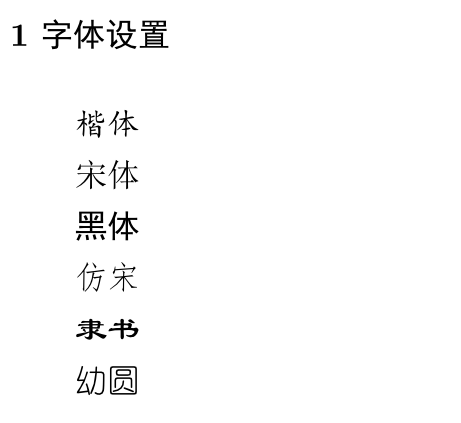使用CJK中文包(GBK格式) \documentclass{article} \usepackage{CJK} \begin{document} \begin{CJK*}{GBK}{song} Hello, World! 世界, 你好! \emph{世界, 你好!} \textbf{世界, 你好!} \end{CJK*} \end{document}  输入中文繁体字 在Windows 10操作系统中，使用微软拼音输入法， 通过 Ctrl + Shift + f 可以转换中文繁/简体 LaTeX文件编译 常用的LaTeX文件编辑软件有WinEdt等，也可以自己动手编译，例如使用Windows的批处理，使用Visual Studio的make等. 使用WinEdt Shift+Ctrl+P Windows 10中使用批处理编译 使用 Windows的 记事本(在文件资源管理器中打开文件夹(如D:\foo)， 点击鼠标右键->新建->文本文档)， 也可以使用别的编译软件如Notepad++等。写入以下命令 pdflatex %1 bibtex %1 pdflatex %1 pdflatex %1 start %1.pdf  保存为bat文件(可以命名为foo.bat) 开始->Windows附件->命令提示符，输入以下命令 cd d:\foo foo.bat  Visual Studio的make 下面是一例用于编译LaTeX文件的Makefile文件: BINPATH = D:\CTEX\MiKTeX\miktex\bin\ TeX =$(BINPATH)\pdflatex.exe
TeX_FLAGS = -shell-escape -interaction=nonstopmode -file-line-error
PRE =  $(TeX) -ini -job-name="preamble" "&pdflatex preamble.tex\dump" BIB =$(BINPATH)\bibtex.exe

FileName = main

all: $(FileName).pdf main.pdf:$(FileName).tex
$(TeX)$(TeX_FLAGS) $(FileName).tex$(BIB) $(FileName).tex$(TeX) $(FileName).tex$(TeX) $(FileName).tex clean: del$(FileName).pdf
del $(FileName).log  常见编译错误 "豢\documentclass{article}" 在Windows系统中，当文档中需要插入中文字符时，需将Latex文件使用UTF-8编码保存。如果使用Windows系统自带的记事本编辑Latex文件，经常遇到下面的编译错误： 豢\documentclass{article}  出现这种编译错误的原因是因为 Windows 会在 UTF 编码的文件最开始加一个 BOM。 解决办法： 不要使用Windows系统自带的记事本保存.tex文件，直接使用别的编辑软件(例如WinEdt, Notepad++等）创建.tex文件。 "Too Many Unprocessed Floats" 如果一浮动对象不能被立即处理，它就会被放到未处理的浮动对象队列@freelist 中, @freelist 一般只保存18个未处理浮动对象，当未处理的浮动对象的数目过多时，就会出现"Too Many Unprocessed Floats"错误。 解决办法： 调整文本 在浮动图形对象之间加入 \clearpage 将浮动图形对象改为非浮动图形对象 使用WinEdt打开UTF-8格式文件，文件的中文字符显示为乱码 解决办法： 在Document->Document Setting->Format->File Format中选择UTF-8格式 或者在在该文件的开始处添加一行 % !Mode:: "TeX:UTF-8"  参考文献  https://en.wikipedia.org/wiki/TeX  http://hubl82.blog.163.com/blog/static/12676948520134593321565/  https://www.shareLaTex.com/learn/Creating_a_document_in_LaTex  http://www.ctex.org/HomePage/  https://www.LaTex-project.org  https://tex.stackexchange.com/questions/246/when-should-i-use-input-vs-include  https://www.sharelatex.com/learn/Bibliography_management_with_bibtex  https://www.verbosus.com/bibtex-style-examples.html  http://www.ctex.org/documents/latex/graphics/node61.html  http://www.ctex.org/documents/latex/graphics/node66.html  http://www.ctex.org/documents/latex/graphics/node87.html#chap:nonfloat  User’s Guide to the Beamer Class. http://www.tuteurs.ens.fr/noncvs/docs/beamer/beameruserguide.pdf  https://www.r-bloggers.com/create-your-own-beamer-template/  https://hamaluik.com/posts/better-beamer-themes/  https://en.wikibooks.org/wiki/LaTeX/Tables  https://www.sharelatex.com/blog/2013/08/27/tikz-series-pt1.html  https://en.wikibooks.org/wiki/LaTeX/Floats,_Figures_and_Captions  https://en.wikibooks.org/wiki/LaTeX/Picture  https://en.wikibooks.org/wiki/LaTeX/Footnotes_and_Margin_Notes  https://en.wikibooks.org/wiki/LaTeX/Boxes  TeX Stack Exchange中的精彩问答 https://www.zhihu.com/question/27240200  The picture Environment http://www.ursoswald.ch/LaTeXGraphics/picture/picture.html  https://tex.stackexchange.com/questions/196794/how-can-you-create-a-vertical-timeline  https://tex.stackexchange.com/questions/74353/what-commands-are-there-for-horizontal-spacing 后记 人类社会历经石器时代, 青铜时代, 铁器时代, 机器时代，电气时代，直到现在的电子信息时代. 人类将对自然界和人类社会探索和研究 转化为知识, 用文字记录下来. 文字的出现标识人类文明的起点. 知识的增长不仅依赖人类对未知世界的探索，也依赖于承载文明的工具. 从结绳刻木, 到龟甲，兽骨, 陶器, 兽皮，竹简, 丝绢, 纸张, 电子计算机, 从刻刀，到笔墨, 活字印刷, 到电子软件, 人类在发现知识的同时也努力改进传承知识的工具. 知识是人类文明的承载体，是人类改变个体命运的强大武器。知识是人类社会划分阶层的重要标尺. 知识曾经是贵族的专享, 二千多年前的孔子提出"有教无类", 打破了贵族对知识的垄断. 感谢那些伟大的程序员. 他(她)们花费时间和精力发明TeX, 开发一系列相关扩展包和辅助工具，将其扩展为LaTex等分支。 他(她)们将私人知识产权的劳动成果奉献为公共财产，供全社会免费使用。 这种大公无私的精神，在物欲横流, 弱肉强食的丛林社会中格外耀眼。他(她)们奉献的不仅是排版软件，更是计算机时代传播知识的工具. 他(她)们无私的奉献使得一些人不再因为金钱而失去获取知识的权力, 也给这些人通过知识改变命运的机会. 这是对人类文明发展的有力推动。 人类文明一直加速发展. 特别从工业革命开始, 出现了牛顿，伏尔泰，康德，达尔文等一大批伟大的思想家, 科学家。在此期间，中国人对人类文明发展做出的贡献于严重落后于我们占世界人口的比重。在国内, 学习LaTex的需求一般源自研究生撰写论文的压力。 能坚持读到这里，你有很大概率是其中的一员。 希望你即使成不了牛顿那样的伟人，至少也要做一个具有奉献精神的人, 能让这个世界变得更美好, 更公平。  展开全文latex • 由于一个项目原因，需要用到R对数据进行回归分析和数据挖掘，因此花半天时间对R进行了一个基础的学习。这是之前整理的网易云课程上的一个视频教程中整理的。 ... ...我刚发现，有可能第一次打开会出现乱码。...  由于一个项目原因，需要用到R对数据进行回归分析和数据挖掘，因此花半天时间对R进行了一个基础的学习。这是之前整理的网易云课程上的一个视频教程中整理的。 这里贴出来，也提供给源代码下载地址（http://pan.baidu.com/s/1c1mV0Xy），方便大家直接在RStudio中执行测试，也方便自己和大家在学习过程中对基本的指令的查询。 我刚发现，有可能第一次打开会出现乱码。这时候在RStudio中选择tools->global options->code->saving里面将text encoding设置为UTF-8就好啦~ 安装RStudio之前必须先安装R，安装包下载地址给分享给大家。 R安装包：http://pan.baidu.com/s/1bnYK2g3 RStudio安装包：http://pan.baidu.com/s/1nu0kvVj 照着敲一遍，包你入门啦~不要偷懒！！不想往下看的话，就去下载上面给出链接的源代码tutorial.R文件哦。 #########################R learning############## ###1.R introduction #### #语言历史和特点 #1.1 R语言历史#### #R是S语言的一种实现。S语言是由AT&T贝尔实验室开发的一种用来进行数据探索、 #统计分析、作图的解释型语言。最初S语言的实现版本主要是S-PLUS. #S-PLUS是一个商业软件，它基于S语言，并由MathSoft公司的统计科学部进一步完善。 #后来Auckland大学的Robert Gentleman和Ross Ihaka及其他志愿人员开发了一个R系统 #R的使用与S-PLUS有很多类似之处，两个软件有一定的兼容性 #1.2 R的特点#### #1.有效地数据处理和保存机制 #2.拥有一整套数组和矩阵的操作运算符 #3.一系列连贯而又完整的数据分析中间工具 #4.图形统计可以对数据直接进行分析和显示，可用于多种图形设备。 #5.一种相当完善、简洁和高效的程序设计语言。包括条件语句、循环语句、用户自定义的递归函数以及输入输出接口。 #6.R语言是彻底面向对象的统计编程语言 #7.R语言和其他编程语言、数据库之间有很好的接口 #8.R语言是自由软件，可以放心大胆地使用，但其功能却不必任何其他同类软件差 #9.R语言具有丰富的网上资源 ###2. Rstudio和R的基本操作#### #2.1查看R语言自带的数据集 data() #直接输入数据集的名称，查看数据 CO2 #2.2快捷键 #Ctrl+Enter： #Ctrl+L： #Ctrl+Shift+S： #Ctrl+1： #Ctrl+2： #Ctrl+D： #2.3查看数据集的前6行#### head(CO2) #查看数据集的最后6行 tail(CO2) #2.4安装包（可以通过命令或者图形界面来咱安装）#### installed.packages("ggplot2") #加载包 library(ggplot2) #2.5创建向量和矩阵以及对它们的基本操作#### x1 <- c(1,2,3,4,5,6) x2 <- c(2,4,6,8,10,12) length(x1) mode(x1) class(x1) rbind(x1,x2) #行联合 cbind(x1, x2) #列联合 Dmat<-cbind(x1,x2) class(Dmat) #使用c函数结合的变量组成的是矩阵 Dmat<-rbind(x1,x2) class(Dmat) Dframe<-as.data.frame(cbind(x1,x2)) class(Dframe) Dmat;Dframe #矩阵和数据框格式是不一样的，矩阵是由行列组成的，数据框是由记录和变量组成的 a<-c(1:100) length(a) cbind(a) a #2.6常用的函数#### b<-c(1:20) mean(b) #均值 sum(b) #求和 max(b) #求最大值 min(b) #求最小值 sd(b) #标准差 #2.7产生向量#### 1:5 1:5*2 1:5*2+1 #2.8查看内存中已有的对象#### ls() #删除当前内存中的所有对象 rm(list=ls()) ls() #2.9访问向量中的元素#### a<-1:5*2-1 a a a[-3] a[2:4] a[-(2:4)] a[1,2,3] #不能这样访问向量中的元素 a[c(1,2,3)] a[a<=5] a[a<=3 | a >= 7] a[a>=3 & a <= 7] a[a] #2.10 seq()函数和rep()函数，用来产生等差数列的函数，调用实例函数#### example("seq") seq(0, 1, length.out = 11) seq(stats::rnorm(20)) # effectively 'along' seq(1, 9, by = 2) # matches 'end' seq(1, 9, by = pi) # stays below 'end' seq(1, 6, by = 3) seq(1.575, 5.125, by = 0.05) seq(17) # same as 1:17, or even better seq_len(17) example(rep) rep(1:4, 2) rep(1:4, each = 2) # not the same. rep(1:4, c(2,2,2,2)) # same as second. rep(1:4, c(2,1,2,1)) rep(1:4, each = 2, len = 4) # first 4 only. rep(1:4, each = 2, len = 10) # 8 integers plus two recycled 1's. rep(1:4, each = 2, times = 3) # length 24, 3 complete replications rep(1, 40*(1-.8)) # length 7 on most platforms rep(1, 40*(1-.8)+1e-7) # better ## replicate a list fred <- list(happy = 1:10, name = "squash") rep(fred, 5) # date-time objects x <- .leap.seconds[1:3] rep(x, 2) rep(as.POSIXlt(x), rep(2, 3)) ## named factor x <- factor(LETTERS[1:4]); names(x) <- letters[1:4] rep(x, 2) rep(x, each = 2) rep.int(x, 2) # no names rep> rep_len(x, 10) #2.11 产生字母序列#### letters[1:30] #2.12 which()函数#### a<-rnorm(10) a which.max(a) #返回数组中最大的数的位置 which.min(a) #返回数组中最小的数的位置 a[which.max(a)] which(a==a[which.max(a)]) which(a>0) a[which(a>0)] #2.13排序函数#### a<-1:10 a rev(a) #反转顺序 a<-c(3,1,5,6,9,2,7,4,6,5) sort(a) rev(sort(a)) #2.14生成矩阵#### a<-c(1:36) a a1<-matrix(a,nrow = 9, ncol = 4);a1 a2<-matrix(a,nrow = 4, ncol = 9);a2 a3<-matrix(a,nrow = 9, ncol = 4, byrow = TRUE);a3 dim(a1) #查看函数的维度 dim(a2) #行 dim(a3) #列 #矩阵运算 t(a1) #转置 a1+a2 a1+a3 #2.15改变RStudio的工作界面（通过tools里面的layout设置完成）#### #2.16 设定工作目录，方便载入数据，将数据存放在设定的目录中，载入数据时就不用指定路径了#### setwd("F:/feiguR/data") setwd("F:\\feiguR\\data") getwd() #用于查看当前工作目录 #2.17 help()函数#### help("seq") ?seq help(package="ggplot2") #2.18 example()函数#### example("seq") example("hist") example("plot") #搜索自己需要的函数 help.search("multivariate normal") #2.19 数据框 dataframe#### ##数据框就是一个数据表格，一行表示一个记录，一列表示一个变量 x1<-c(1,2,3,4,5,6,7,8,9) x2<-c(2,4,6,8,10,12,14,16,18) x<-data.frame(x1,x2);x x=data.frame('weight'=x1, 'cost'=x2);x x$"feerate"<-x$cost/x$weight    #数据框可以利用已有的变量产生新的变量并存储于当前数据框中
names(x)   #查看数据框中的变量
str(x)    #查看数据框中数据的定义
#我们在载入数据后，要使用上面的两个函数来检查数据载入是否成功，并检查数据的定义
#2.20 列表list() ####
x1<-1  #单个数字，其实是一个只有一个元素的一维向量
x2<-c(1,2,3,4)
x3<-c("a","bc","d")    #字符向量
x4<-matrix(1:36,nrow = 9,ncol = 4)    #矩阵，vector
x5<-data.frame(a=c(1,2,3,4), b=c(2,3,4,5),c=c(3,4,5,6))
list_01<-list(x1=x1,x2=x2,x3=x3,x4=x4,x5=x5)     #产生一个数据框
list_01$x3 ###3. 数据导入与导出 #### #3.1 先把R内置的CO2数据集导出，然后再联系导入操作#### write.table(CO2,file="二氧化碳.txt") write.csv(CO2,file = "二氧化碳.csv") #3.2 导入逗号分割的文本文件#### read.table(file = "二氧化碳.txt", header = TRUE) #最好指定一个名称 carbon<-read.table(file = "二氧化碳.txt", header = TRUE) #3.3 导入csv格式的数据#### carbon_csv<-read.csv(file = "二氧化碳.csv", header = TRUE) #3.4通过剪贴板读入数据并写入文件 setwd("F:/feiguR") clipboard<-read.table("clipboard",header = TRUE) clipboard write.table(clipboard, file = "clipboard.txt") #3.5 导入空格分割的文本文件数据#### setwd("F:/feiguR") lowtem<-read.table(file = "lowtemperature.prn", header = TRUE) names(lowtem);str(lowtem) lowtem$fyear<-factor(lowtem$year, c(2013,2014,2015), labels = c("2013年","2014年","2015年")) #此处设计重新编码变量的操作 #3.5.1 读取数据框中的变量#### #1）很多函数中有data参数，可以指定数据框，然后在函数内部直接访问数据框中的变量 boxplot(lowtem~year, data = lowtem) #2)使用美元符号$
boxplot(lowtem$lowtem~lowtem$year)
#3)使用attach函数，不推荐使用，很容易出错，特别是在同一个R代码及中写很多不同程序的时候
attach(lowtem)
boxplot(lowtem~year)
detach(lowtem)
###3.6 数据子集操作####
#1)先明确分类变量有几个类别
unique(lowtem$year) #2)选择数据子集 lowtem_15<-lowtem[lowtem$year=2015,]
plot.ts(lowtem_15$lowtem) #3)多个条件用逻辑连接符号进行连接 lowtem_1501<-lowtem[lowtem$year==2015 & lowtem$day=="1/1",] lowtem_1501 #4)排序操作 #按照日最低气温进行排序，这个排序可能没有实际意义，这里只是演示操作的方便 orderlowtem<-lowtem[order(lowtem$lowtem),]    #对行的操作
View(orderlowtem)
#5)合并数据子集
#加入三年的气温数据是分开的，我们要将它们合并到一个数据集中
#（1）先读入数据
setwd("F:/feiguR")
write.table(hightem, file = "hightem.txt", quote = FALSE)  #将这个数据保存一边下次使用
#(2)然后进行合并
colnames(hightem)<-c("day","year","hightem")  #由于变量名称大小写不一致，对最高温度的数据集变量名称进行重命名
tem<-merge(hightem, lowtem, by=c("year", "day"))
tem$day<-as.Date(tem$day)
#(3)合并后有一个问题就是时间序列被打乱了
#暂时解决办法是把文件写入csv文件，用excel重新编辑日期，并排序后重新导入
#3.7 读入excel格式的文件####
install.packages("RODBC")  #注意有些包只在特定版本中能够运行，更新问题
library(RODBC)
xls<-odbcConnectExcel("wechat.xls")  #odbcConnectExcel is only usable with 32-bit Windows
###4. 常用的函数####
##4.1 tapply函数（用来对某数值变量依据另外一个分类变量求某值）####
setwd("F:/feiguR")
str(tem_new)
tapply(tem_new$lowtem, tem_new$year, mean)  #计算最低气温均值
tapply(tem_new$hightem, tem_new$year, mean)  #计算最高气温均值
tapply(tem_new_lowtem, tem_new$year, sd) #计算最低气温方差 ###4.2 sapply()函数 和 lapply()函数（对多个变量求某函数的结果）#### sapply(tem_new[,4:5], mean) #输出为向量 lapply(tem_new[,4:5], mean) #输出为列表 ##4.3 summary()函数 用来输出汇总信息#### a<-summary(tem_new) b<-summary(tem_new$year)
c<-summary(c(1,2,3,4,5,6,7,8,9))
d<-lm(tem_new$hightem~tem_new$lowtem)
e<-summary(d)
class(a);class(b);class(c);class(d);class(e)   #summary函数的输出非常丰富，是一种列表
e$coeeficients ###4.4. table函数 (用于单变量的频数统计，多分类变量的交叉分析)#### example("table") #4.4.1 简单的频数统计#### rpois(100,5) table(rpois(100,5)) #4.4.2 查看表的结构（可用于对数据框中两个分类变量进行交叉分析）#### View(warpbreaks) with(warpbreaks, table(wool, tension)) ###5. 绘图工具 #### #5.1 基本绘图函数plot() #### View(cars) help(cars) #速度与刹车距离 head(cars) plot(cars) plot(cars$dist~cars$speed) #指定y轴表示刹车距离 plot(cars$speed~cars$dist) #指定x轴表示刹车距离 #5.2 为了方便将两幅图在一起进行对比#### windows() #用单独的窗口显示图形以获得更好的显示效果，注意windows命令在前 par(mfrow=c(2,2)) plot(cars$dist~cars$speed) plot(cars$speed~cars$dist) #5.3函数的参数#### plot(cars$dist~cars$speed, #y~x main="刹车距离与车速之间的关系", #画标题 xlab="Speed(miles per hour)", #X坐标轴标题 ylab = "Distance travelled(miles)", #y坐标轴标题 xlim = c(0,30), #x坐标轴范围 ylim = c(0,140), #y坐标轴范围 xaxs = "i", #设置x轴风格为internal yaxs = "i", #设置y轴风格为internal col = "red", #设置散点的颜色为红色 pch = 19 #设置散点的形状为实心圆点 ) #5.4 绘制线图 #### #使用微信公众号数据 setwd("...") num<-read.csv(file = "wechat.csv") head(num) windows() plot(num$NetGrowth~as.Date(num$date), type="1", main="每日净增长变化", xlab="date", ylab="每日净增长人数", col = "red" ) ##5.5 低水平绘图函数lines() #### lines(num$NetGrowth~as.Date(num$date),col = "red") example("lines") plot(cars, main = "Stopping Distance versus Speed") lines(stats::lowess(cars)) #此代码为散点图加上光滑的曲线 #5.6 柱形图#### #使用数据集BOD View(BOD) #使用基础绘图包 barplot(BOD$demand, names.arg = BOD$Time) #先输出频数表，然后在用条形图绘制 table(mtcars$cyl)
barplot(table(mtcars$cyl)) #改变图形的方向 windows() par(mfrow=c(1,2)) barplot(BOD$demand, names.arg = BOD$Time) barplot(BOD$demand, names.arg = BOD$Time, horiz = TRUE) #5.7 绘制直方图#### windows() par(mfrow=c(1,2)) hist(mtcars$mpg)
hist(mtcars$mpg, breaks = 10) #控制分区数目 #5.8 绘制箱线图#### #使用数据集ToothGrowth View(ToothGrowth) #使用plot函数时，当x轴为分类变量，y轴为数值型变量时，默认输出箱线图 plot(ToothGrowth$supp, ToothGrowth$len) #5.9 绘图设备（输出图形的格式）#### ?device setwd("F:/feiguR") pdf("fly.pdf") #在图形设备中输出图形名称 plot(ToothGrowth$supp, ToothGrowth$len) dev.off() #关闭当前图形设备，相当于保存该图形 png("fly.png") plot(ToothGrowth$supp, ToothGrowth$len) dev.off() pdf("xxx.pdf") #在你希望将所有图形输出到一个文件中时，这样做不支持PNG格式 hist(mtcars$mpg)
hist(mtcars$mpg, breaks = 10) plot(ToothGrowth$supp, ToothGrowth$len) dev.off() #图形设备设置 dev.cur() #查看当前正在使用的图形设备 dev.set(3) #把当前正在使用的图形设备设置为其他设备 #5.10 其他常用图形#### example("pie") #查看饼图的使用方法和实例 example("plot.ts") #5.11 绘图参数#### setwd("F:/feiguR") wec<-read.csv(file = "wechat.csv", header = TRUE) head(wec) windows() plot.ts(wec$NetGrowth, col="red")
points(wec$NetGrowth, bg="skyblue", color="red", pch=21) abline(h=mean(wec$NetGrowth), col="blue")
title("公众号日增净关注人数变化")
box()
#5.12 生成时间序列的函数####
ts(1:10, frequency = 4, start = c(1959,2))   #生成时间序列，季度，起始
print(ts(1:10, frequency = 7, start = c(12,2)), calendar = TRUE)    #星期 日历
gnp<- ts(cumsum(1+round(rnorm(100),2)), start = c(1954,7), frequency = 12)
z <- ts(matrix(rnorm(300), 100, 3), start = c(1961,1), frequency = 12)
class(z)
plot(z)
###6. R语言与统计####
###7. 循环与自定义函数####
#7.1 for循环####
example("for")
for (i in 1:5) {     #循环遍历每个数，每取出一个数就打印一个向量
print(1:i)
}
for (n in c(2,5,10,20,50)) {
x <- stats::rnorm(n)    #生成含有n个数的正态分布
cat(n, ":", sum(x^2), "\n")  #对应每一个数，计算生成的正太分布数组的平方和
}
f<-factor(sample(letters[1:5], 10, replace = TRUE));f    #抽样，生成字母序列
for (i in unique(f)) {  #依次去除序列中的每一个独有的值
print(i)
}
#7.2 while语句####
a<-1; i<-1
while(a[i] < 100){
i = i + 1;
a[i] <- a[i-1]+3
}
#7.3 自定义函数####
myfunc_cv<-function(x){   #函数在R中也是一个对象
cv<-sd(x)/mean(x)
return(cv)       #变异系数
}
#测试该函数
a<-c(1,2,5,8,9,6)
myfunc_cv(a)
#7.4 通过循环和自定义函数来验证中心极限定理####
myfun<-function(a){
x<-1:100   #先生成一个1到100的序列，后面可以更改这些值，相当于覆盖掉原来的值
x<-data.frame(x)
a<-data.frame(a)

for (i in 1:100) {     #设置循环，循环抽取100个样本，并将计算出来的均值付给数据框中的x变量
c<-a[sample(nrow(a), 1000),]
m = mean(c)
x$x[i] = m } windows(1280,720);par(mfrow=c(1,2)) plot(density(a$a),main="这是原来的分布")
plot(density(x\$x), main = "这是抽取的样本的均数的分布")
}
a<-c(1:1000)
myfun(a)
###7.4.1 正态分布####
a<-rnorm(10000,0,1)
myfun(a)
###7.4.2 指数分布####
b<-rexp(10000,1)
myfun(b)
###7.4.3 t分布####
c<-rt(1000,3)
myfun(c)
###7.4.4 F分布####
d<-rchisq(100000,1)
myfun(d)
###8.数据处理####
###8.1 把数据框变长####
其中的条目最后的四个'#'号是做成目录的一个代码（必须只能是四个‘#’号），在RStudio中可以直接在代码编辑框下面选择。
`
展开全文• 入门到大神，都可以在Kaggle找到适合自己的竞赛。 常见的竞赛类型如下，后面有具体的介绍。 a）入门，官方为新手入门准备的三个小项目，有提供答案。 b）游乐场，新手进阶练习项目，提高技能。 c）精选，...Kaggle
• Atom是GitHub推出的一款编辑器,被称为21世纪的黑客编辑器,主要的特点是现代,易用,可定制.
• C语言入门 -&gt; Linux C语言编程基本原理与实践 -&gt; Linux C语言指针与内存 -&gt; Linux C语言结构体 https://www.imooc.com/course/programdetail/pid/37 c语言入门 C语言一经出现就以其功能...C 编程语言
• 五一这两天花了点时间把自己有道电子笔记上过去写的东西给整理了一下，因为重装了系统之后大部分以前做的笔记和写的文章都丢了，于是想想还是把没丢的这些给整理一下放在博客了为好！Express是流行的Node.js Web框架...Express
• 已经火了很久了，一直想了解它学习它结果没时间，了解了一些资料，结合我自己的情况，整理了一个学习路线。     ...想要在大数据这个领域汲取养分，让自己壮大成长。分享方向，行动以前先分享下一个大数据交流...大数据 大数据学习 大数据开发 人工智能 程序员
• ## 入门

2018-08-25 10:17:18
入门 Description 不是任何人都可以进入桃花岛的，黄药师最讨厌象郭靖一样呆头呆脑的人。所以，他在桃花岛的唯一入口处修了一 条小路，这条小路全部用正方形瓷砖铺设而成。有的瓷砖可以踩，我们认为是安全的，而...
• 软件测试入门视频培训教程：该课程将带你走进“软件测试”的大门，具体内容包括软件测试环境搭建、软件开发模型、产品模型、CMM模型、测试用例、等价类划分、边界值划分、白盒测试、单元测试、bugfree搭建、系统测试...软件测试 软件测试面试题 软件测试面试 软件测试需要学什么 软件测试简历
• 教程会把 C语言入门 的核心内容做关键知识点的提纯，去除一些笼统的废话，配以思维导图与经典例子， 相信可以让初学者更快的上手C语言。另外笔者只是大学学过下C基础的东西， 不是专业选手，如果本教程有什么纰漏...c语言 快速 入门 新手 教程
• 小猪的C语言快速入门系列(四)标签： C语言本节引言：经过前面三节的学习，我们对于C语言有了一点基本的了解， 假如现在要你根据下面的题目写一个这样的代码应该不难吧：题目： 输入五个学生的成绩，然后求出总和和...c语言 新手 入门 快速 教程
• 今天心血来潮给大家写个新手到黑客入门的路径图【附全部学习资料下载】！ 入门介绍： 说到黑客大家可能觉得很神秘，其实我们说的的黑客是白帽子黑客，就是去寻找网站、系统、软件等漏洞并帮助厂商修复的人，刚入门...
• 小猪的C语言快速入门系列(七)标签： C语言本节引言：在第五节的时候我们对C里的指针进行了初步的学习，而作为C语言的灵魂， 当然不会就那么简单，本节我们来进一步学习指针，包括：函数与指针， 指针数组，二级...C语言 入门 快速 新手 教程
• 小猪的C语言快速入门系列(三)标签： C语言本节引言：在上一节中，对C语言的基本语法进行了学习，类比成学英语的话，我们现在 只是会单词而已，组成一个个句子还需要学习一些语法，本节学习的就是两对 输入输出函数...C语言 快速 入门 新手 教程
...